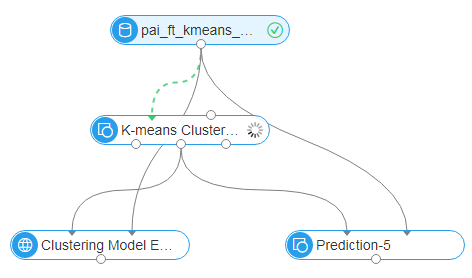Search
Document Center

# Machine learning

Last Updated: Jun 01, 2020

## Linear SVM

Developed in the mid 90’s, Support Vector Machine (SVM) is a statistical learning theory based machine learning method. It seeks to improve the learning machine’s generalization ability through structural risk minimization, so as to minimize the empirical risk and confidence range. Therefore, good statistics can be obtained from small sample sizes. For more information about SVM, see wiki.

This linear SVM is not implemented using the kernel function. For details about the implementation, see section “6 Trust Region Method for L2-SVM” in http://www.csie.ntu.edu.tw/~cjlin/papers/logistic.pdf. This algorithm only supports binary classification models.

#### Algorithm component

1. Set field parameters of the component.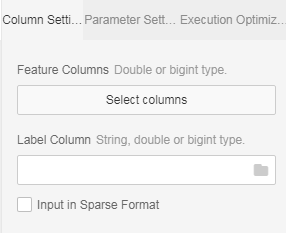• Input column: You can select only a column of bigint or double type.

• Label column: The data type of the label column can be bigint, double, or string. This component supports only binary classification models.

2. Set algorithm parameters.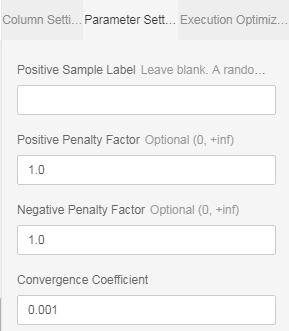• Penalty factor: Defaults to 1.

• Base value: (Optional) Positive value. If this parameter is not specified, the system selects a random value. We recommend that you specify this parameter when the positive and negative samples are significantly different.

• Positive weight: (Optional) Positive penalty factor. The default value is 1.0, and the value range is (0, ~).

• Negative weight: (Optional) Negative penalty factor. The default value is 1.0, and the value range is (0, ~).

• Convergence coefficient: (Optional) Convergence deviation. The default value is 0.001, and the value range is (0, 1).

NOTE: If the base value is not specified, the positive weight and negative weight must be the same.

#### PAI command

``PAI -name LinearSVM -project algo_public ``    -DnegativeCost="1.0" \``    -DmodelName="xlab_m_LinearSVM_6143"  ``    -DpositiveCost="1.0" \``    -Depsilon="0.001" ``    -DlabelColName="y" \``    -DfeatureColNames="pdays,emp_var_rate,cons_conf_idx" \``    -DinputTableName="bank_data" ``    -DpositiveLabel="0";``

#### Parameter settings

Parameter Description Option Default value
inputTableName Name of the input table NA NA
inputTableParitions (Optional) Partitions used for training in the input table, in the format of Partition_name=value. The multilevel format is name1=value1/name2=value2, multiple partitions are separated by commas NA All partitions in the input table
modelName (Required) Name of the output model NA NA
featureColNames (Required) Names of the feature columns used for training in the input table NA NA
labelColName (Required) Name of the label column in the input table NA NA
positiveLabel (Optional) Positive value NA Random value selected among label values
negativeCost (Optional) Negative weight (negative penalty factor) (0, ∞) 1.0
positiveCost (Optional) Positive weight (positive penalty factor) (0, ∞) 1.0
(Optional) Convergence coefficient (0, 1) 0.001

#### Example

Training data

id y f0 f1 f2 f3 f4 f5 f6 f7
1 -1 -0.294118 0.487437 0.180328 -0.292929 -1 0.00149028 -0.53117 -0.0333333
2 +1 -0.882353 -0.145729 0.0819672 -0.414141 -1 -0.207153 -0.766866 -0.666667
3 -1 -0.0588235 0.839196 0.0491803 -1 -1 -0.305514 -0.492741 -0.633333
4 +1 -0.882353 -0.105528 0.0819672 -0.535354 -0.777778 -0.162444 -0.923997 -1
5 -1 -1 0.376884 -0.344262 -0.292929 -0.602837 0.28465 0.887276 -0.6
6 +1 -0.411765 0.165829 0.213115 -1 -1 -0.23696 -0.894962 -0.7
7 -1 -0.647059 -0.21608 -0.180328 -0.353535 -0.791962 -0.0760059 -0.854825 -0.833333
8 +1 0.176471 0.155779 -1 -1 -1 0.052161 -0.952178 -0.733333
9 -1 -0.764706 0.979899 0.147541 -0.0909091 0.283688 -0.0909091 -0.931682 0.0666667
10 -1 -0.0588235 0.256281 0.57377 -1 -1 -1 -0.868488 0.1

Test data

id y f0 f1 f2 f3 f4 f5 f6 f7
1 +1 -0.882353 0.0854271 0.442623 -0.616162 -1 -0.19225 -0.725021 -0.9
2 +1 -0.294118 -0.0351759 -1 -1 -1 -0.293592 -0.904355 -0.766667
3 +1 -0.882353 0.246231 0.213115 -0.272727 -1 -0.171386 -0.981213 -0.7
4 -1 -0.176471 0.507538 0.278689 -0.414141 -0.702128 0.0491804 -0.475662 0.1
5 -1 -0.529412 0.839196 -1 -1 -1 -0.153502 -0.885568 -0.5
6 +1 -0.882353 0.246231 -0.0163934 -0.353535 -1 0.0670641 -0.627669 -1
7 -1 -0.882353 0.819095 0.278689 -0.151515 -0.307329 0.19225 0.00768574 -0.966667
8 +1 -0.882353 -0.0753769 0.0163934 -0.494949 -0.903073 -0.418778 -0.654996 -0.866667
9 +1 -1 0.527638 0.344262 -0.212121 -0.356974 0.23696 -0.836038 -0.8
10 +1 -0.882353 0.115578 0.0163934 -0.737374 -0.56974 -0.28465 -0.948762 -0.933333
1. Create an experiment svm_example.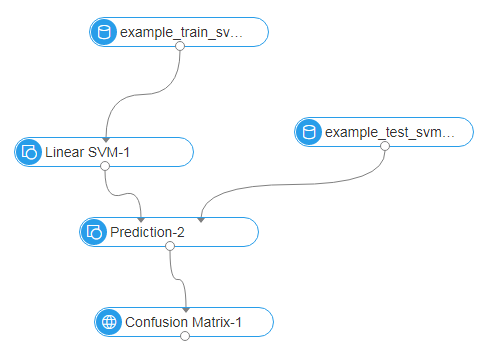1. Select feature columns.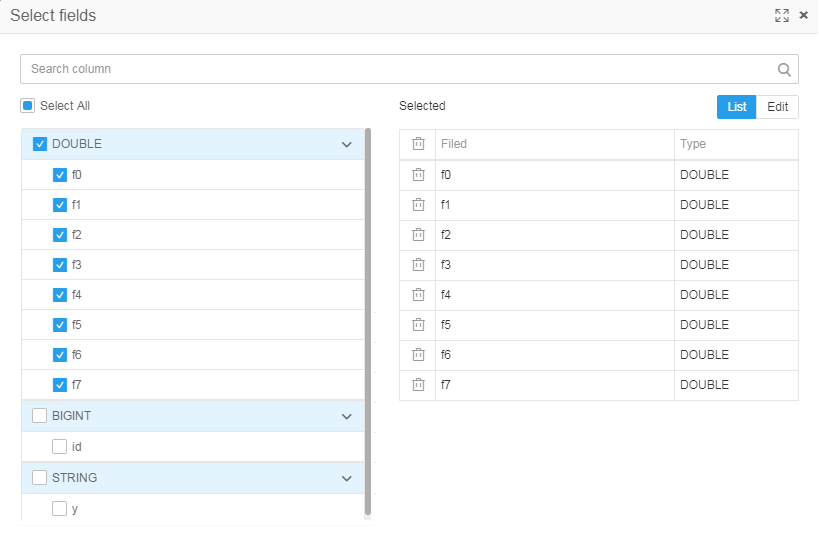2. Select the label column.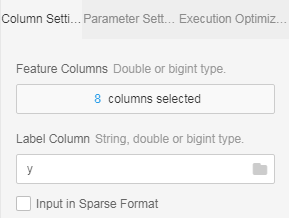3. Set SVM parameters.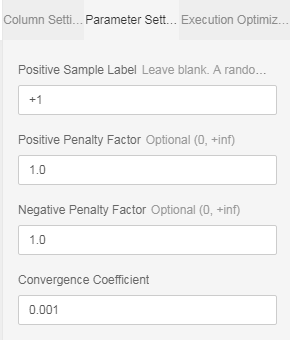4. Run the experiment.

• The following model is generated: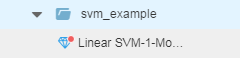• The following figure shows the predicted result.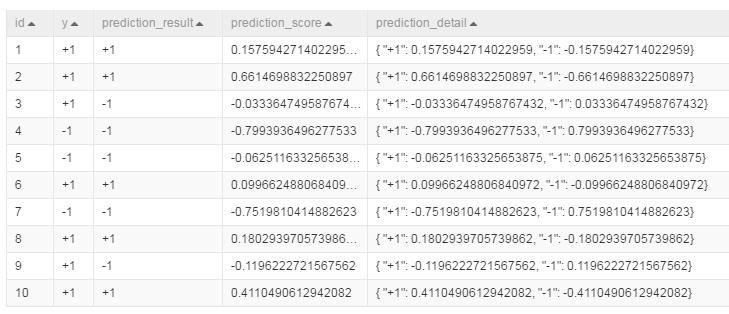## Logistic regression

• Classic logistic regression is a binary classification algorithm. Logistic regression on the algorithm platform supports multiclass classification.

• The logistic regression component supports two data formats: sparse and dense.

• Logical regression for multiclass classification supports a maximum of 100 classes.

#### Parameter settings

Parameters of the logistic regression component:

• Support for sparse matrix: The component supports the sparse matrix format.

• Base value: (Optional) Specify the label value of the training coefficient in the case of binary classification. If this parameter is left blank, the system selects a random value.

• Maximum iterations: (Optional) Maximum number of L-BFGS iterations. The default value is 100.

• Convergence deviation: (Optional) Condition for L-BFGS termination, that is, the log-likelihood deviation between two iterations. The default value is 1.0e-06.

• Regularization type: (Optional) Options are l1’, ‘l2’, and ‘None’. The default value is ‘l1’.

• Regularization coefficient: (Optional) The default value is 1.0. This parameter is ignored when regularizedType is set to None.

#### PAI command (Not inheriting the type setting node)

``PAI -name LogisticRegression -project algo_public ``    -DmodelName="xlab_m_logistic_regression_6096" \``    -DregularizedLevel="1" ``    -DmaxIter="100" ``    -DregularizedType="l1" ``    -Depsilon="0.000001" ``    -DlabelColName="y"\``    -DfeatureColNames="pdays,emp_var_rate" ``    -DgoodValue="1" ``    -DinputTableName="bank_data";``
• name: component name.

• project: Name of the project. This parameter specifies the space where an algorithm is located. The default value is algo_public. If you change the default value, the system returns an error.

• modelName: Name of the output model.

• regularizedLevel: (Optional) Regularization coefficient. The default value is 1.0. This parameter is ignored if regularizedType is set to None.

• maxIter: (Optional) Maximum iterations. It specifies the maximum number of L-BFGS iterations. The default value is 100.

• regularizedType: (Optional) Regularization type. Options are l1’, ‘l2’, and ‘None’. The default value is ‘l1’.

• epsilon: (Optional) Convergence deviation. It is the condition for L-BFGS termination, that is, the log-likelihood deviation between two iterations. The default value is 1.0e-06.

• labelColName: Name of the label column in the input table.

• featureColNames: Names of the feature columns used for training in the input table.

• goodValue: (Optional) Base value. For binary classification, specify the label value of the training coefficient. If this parameter is left blank, the system selects a random value.

• inputTableName: Name of the input table for training.

#### Example

##### Binary classification

Test data

SQL statement for data generation

``drop table if exists lr_test_input;``create table lr_test_input``as``select``    *``from``(``    select``        cast(1 as double) as f0,``        cast(0 as double) as f1,``        cast(0 as double) as f2,``        cast(0 as double) as f3,``        cast(0 as bigint) as label``    from dual``    union all``        select``            cast(0 as double) as f0,``            cast(1 as double) as f1,``            cast(0 as double) as f2,``            cast(0 as double) as f3,``            cast(0 as bigint) as label``    from dual``    union all``        select``            cast(0 as double) as f0,``            cast(0 as double) as f1,``            cast(1 as double) as f2,``            cast(0 as double) as f3,``            cast(1 as bigint) as label``    from dual``    union all``        select``            cast(0 as double) as f0,``            cast(0 as double) as f1,``            cast(0 as double) as f2,``            cast(1 as double) as f3,``            cast(1 as bigint) as label``    from dual``    union all``        select``            cast(1 as double) as f0,``            cast(0 as double) as f1,``            cast(0 as double) as f2,``            cast(0 as double) as f3,``            cast(0 as bigint) as label``    from dual``    union all``        select``            cast(0 as double) as f0,``            cast(1 as double) as f1,``            cast(0 as double) as f2,``            cast(0 as double) as f3,``            cast(0 as bigint) as label``    from dual``) a;``

Input data description

``+------------+------------+------------+------------+------------+``| f0         | f1         | f2         | f3         | label      |``+------------+------------+------------+------------+------------+``| 1.0        | 0.0        | 0.0        | 0.0        | 0          |``| 0.0        | 0.0        | 1.0        | 0.0        | 1          |``| 0.0        | 0.0        | 0.0        | 1.0        | 1          |``| 0.0        | 1.0        | 0.0        | 0.0        | 0          |``| 1.0        | 0.0        | 0.0        | 0.0        | 0          |``| 0.0        | 1.0        | 0.0        | 0.0        | 0          |``+------------+------------+------------+------------+------------+``

Running command

``drop offlinemodel if exists lr_test_model;``drop table if exists lr_test_prediction_result;``PAI -name logisticregression_binary -project algo_public -DmodelName="lr_test_model" -DitemDelimiter="," -DregularizedLevel="1" -DmaxIter="100" -DregularizedType="None" -Depsilon="0.000001" -DkvDelimiter=":" -DlabelColName="label" -DfeatureColNames="f0,f1,f2,f3" -DenableSparse="false" -DgoodValue="1" -DinputTableName="lr_test_input";``PAI -name prediction -project algo_public -DdetailColName="prediction_detail" -DmodelName="lr_test_model" -DitemDelimiter="," -DresultColName="prediction_result" -Dlifecycle="28" -DoutputTableName="lr_test_prediction_result" -DscoreColName="prediction_score" -DkvDelimiter=":" -DinputTableName="lr_test_input" -DenableSparse="false" -DappendColNames="label";``

Running result

lr_test_prediction_result

``+------------+-------------------+------------------+-------------------+``| label      | prediction_result | prediction_score | prediction_detail |``+------------+-------------------+------------------+-------------------+``| 0          | 0                 | 0.9999998793434426 | {``    "0": 0.9999998793434426,``        "1": 1.206565574533681e-07} |``        | 1          | 1                 | 0.999999799574135 | {``            "0": 2.004258650156743e-07,``                "1": 0.999999799574135} |``                | 1          | 1                 | 0.999999799574135 | {``                    "0": 2.004258650156743e-07,``                        "1": 0.999999799574135} |``                        | 0          | 0                 | 0.9999998793434426 | {``                            "0": 0.9999998793434426,``                                "1": 1.206565574533681e-07} |``                                | 0          | 0                 | 0.9999998793434426 | {``                                    "0": 0.9999998793434426,``                                        "1": 1.206565574533681e-07} |``                                        | 0          | 0                 | 0.9999998793434426 | {``                                            "0": 0.9999998793434426,``                                                "1": 1.206565574533681e-07} |``                                                +------------+-------------------+------------------+-------------------+``
##### Multiclass classification

Test data

SQL statement for data generation

``drop table if exists multi_lr_test_input;``create table multi_lr_test_input``as``select``    *``from``(``    select``        cast(1 as double) as f0,``        cast(0 as double) as f1,``        cast(0 as double) as f2,``        cast(0 as double) as f3,``        cast(0 as bigint) as label``    from dual``    union all``        select``            cast(0 as double) as f0,``            cast(1 as double) as f1,``            cast(0 as double) as f2,``            cast(0 as double) as f3,``            cast(0 as bigint) as label``    from dual``    union all``        select``            cast(0 as double) as f0,``            cast(0 as double) as f1,``            cast(1 as double) as f2,``            cast(0 as double) as f3,``            cast(2 as bigint) as label``    from dual``    union all``        select``            cast(0 as double) as f0,``            cast(0 as double) as f1,``            cast(0 as double) as f2,``            cast(1 as double) as f3,``            cast(1 as bigint) as label``    from dual``) a;``

Input data description

``+------------+------------+------------+------------+------------+``| f0         | f1         | f2         | f3         | label      |``+------------+------------+------------+------------+------------+``| 1.0        | 0.0        | 0.0        | 0.0        | 0          |``| 0.0        | 0.0        | 1.0        | 0.0        | 2          |``| 0.0        | 0.0        | 0.0        | 1.0        | 1          |``| 0.0        | 1.0        | 0.0        | 0.0        | 0          |``+------------+------------+------------+------------+------------+``

Running command

``drop offlinemodel if exists multi_lr_test_model;``drop table if exists multi_lr_test_prediction_result;``PAI -name logisticregression_multi -project algo_public -DmodelName="multi_lr_test_model" -DitemDelimiter="," -DregularizedLevel="1" -DmaxIter="100" -DregularizedType="None" -Depsilon="0.000001" -DkvDelimiter=":" -DlabelColName="label" -DfeatureColNames="f0,f1,f2,f3" -DenableSparse="false" -DinputTableName="multi_lr_test_input";``PAI -name prediction -project algo_public -DdetailColName="prediction_detail" -DmodelName="multi_lr_test_model" -DitemDelimiter="," -DresultColName="prediction_result" -Dlifecycle="28" -DoutputTableName="multi_lr_test_prediction_result" -DscoreColName="prediction_score" -DkvDelimiter=":" -DinputTableName="multi_lr_test_input" -DenableSparse="false" -DappendColNames="label";``

Running result

multi_lr_test_prediction_result

``+------------+-------------------+------------------+-------------------+``| label      | prediction_result | prediction_score | prediction_detail |``+------------+-------------------+------------------+-------------------+``| 0          | 0                 | 0.9999997274902165 | {``    "0": 0.9999997274902165,``        "1": 2.324679066261573e-07,``            "2": 2.324679066261569e-07} |``            | 0          | 0                 | 0.9999997274902165 | {``                "0": 0.9999997274902165,``                    "1": 2.324679066261573e-07,``                        "2": 2.324679066261569e-07} |``                        | 2          | 2                 | 0.9999999155958832 | {``                            "0": 2.018833979850994e-07,``                                "1": 2.324679066261573e-07,``                                    "2": 0.9999999155958832} |``                                    | 1          | 1                 | 0.9999999155958832 | {``                                        "0": 2.018833979850994e-07,``                                            "1": 0.9999999155958832,``                                                "2": 2.324679066261569e-07} |``                                                +------------+-------------------+------------------+-------------------+``

## GBDT binary classification

This component is used to resolve binary classification issues based on GBDT regression and sorting. Values greater than the preset threshold are positive values and those smaller than the threshold are negative values.

#### PAI command

``PAI -name gbdt_lr``    -project algo_public``    -DfeatureSplitValueMaxSize="500"``    -DrandSeed="0" ``    -Dshrinkage="0.5"``    -DmaxLeafCount="32"``    -DlabelColName="y"``    -DinputTableName="bank_data_partition" ``    -DminLeafSampleCount="500"``    -DgroupIDColName="nr_employed"``    -DsampleRatio="0.6"``    -DmaxDepth="11" ``    -DmodelName="xlab_m_GBDT_LR_21208"``    -DmetricType="2"``    -DfeatureRatio="0.6"``    -DinputTablePartitions="pt=20150501"``    -DtestRatio="0.0"``    -DfeatureColNames="age,previous,cons_conf_idx,euribor3m"``    -DtreeCount="500"``

#### Parameter description

Parameter Description Value range Required/Optional, default value
inputTableName Input table Table name Required
featureColNames Names of the feature columns used for training in the input table Column names Optional, default: all columns with values
labelColName Name of the label column in the input table Column name Required
inputTablePartitions Partitions used for training in the input table, in the format of partition_name=value. The multilevel format is name1=value1/name2=value2. Multiple partitions are separated by commas (,). NA (Optional) All partitions are selected by default.
modelName Name of the output model NA Required
outputImportanceTableName Name of the output feature importance table NA Optional
groupIDColName Name of a grouping column Column name Optional, default: full table
lossType Loss function type, 0: GBRANK, 1: LAMBDAMART_DCG, 2: LAMBDAMART_NDCG, 3: LEAST_SQUARE, 4: LOG_LIKELIHOOD 0, 1, 2, 3, 4 Optional, default: 0
metricType Metric type, 0(NDCG)-: normalized discounted cumulative gain; 1(DCG): discounted cumulative gain; 2 (AUC) adaptive only to 0/1 label 0, 1, 2 Optional, default: 2
treeCount Number of trees [1, 10,000] Optional, default: 500
shrinkage Learning rate (0, 1] Optional, default: 0.05
maxLeafCount Maximum number of leaves, which must be an integer [2, 1000] Optional, default: 32
maxDepth Maximum depth of a tree, which must be an integer [1, 11] Optional, default: 11
minLeafSampleCount Minimum number of samples on a leaf node, which must be an integer [100, 1000] Optional, default: 500
sampleRatio Ratio of samples collected during the training (0, 1] Optional, default: 0.6
featureRatio Ratio of features collected during the training (0, 1] Optional, default: 0.6
tau Parameter Tau in gbrank loss [0, 1] Optional, default: 0.6
p Parameter p in gbrank loss [1, 10] Optional, default: 1
randSeed Random seed [0, 10] Optional, default: 0
newtonStep Whether to use the newton method 0,1 Optional, default: 1
featureSplitValueMaxSize Number of records that a feature can split into [1, 1000] Optional, default: 500
lifecycle Lifecycle of the output table NA Optional, default: not set

#### Example

Data generation

``drop table if exists gbdt_lr_test_input;``create table gbdt_lr_test_input``as``select``    *``from``(``    select``        cast(1 as double) as f0,``        cast(0 as double) as f1,``        cast(0 as double) as f2,``        cast(0 as double) as f3,``        cast(0 as bigint) as label``    from dual``    union all``        select``            cast(0 as double) as f0,``            cast(1 as double) as f1,``            cast(0 as double) as f2,``            cast(0 as double) as f3,``            cast(0 as bigint) as label``    from dual``    union all``        select``            cast(0 as double) as f0,``            cast(0 as double) as f1,``            cast(1 as double) as f2,``            cast(0 as double) as f3,``            cast(1 as bigint) as label``    from dual``    union all``        select``            cast(0 as double) as f0,``            cast(0 as double) as f1,``            cast(0 as double) as f2,``            cast(1 as double) as f3,``            cast(1 as bigint) as label``    from dual``    union all``        select``            cast(1 as double) as f0,``            cast(0 as double) as f1,``            cast(0 as double) as f2,``            cast(0 as double) as f3,``            cast(0 as bigint) as label``    from dual``    union all``        select``            cast(0 as double) as f0,``            cast(1 as double) as f1,``            cast(0 as double) as f2,``            cast(0 as double) as f3,``            cast(0 as bigint) as label``    from dual``) a;``

PAI command

Training

``drop offlinemodel if exists gbdt_lr_test_model;``PAI -name gbdt_lr``    -project algo_public``    -DfeatureSplitValueMaxSize="500"``    -DrandSeed="1"``    -Dshrinkage="1"``    -DmaxLeafCount="30"``    -DlabelColName="label"``    -DinputTableName="gbdt_lr_test_input"``    -DminLeafSampleCount="1"``    -DsampleRatio="1"``    -DmaxDepth="10"``    -DmodelName="gbdt_lr_test_model"``    -DmetricType="0"``    -DfeatureRatio="1"``    -DtestRatio="0"``    -DfeatureColNames="f0,f1,f2,f3"``    -DtreeCount="5"``

Prediction

``drop table if exists gbdt_lr_test_prediction_result;``PAI -name prediction``    -project algo_public``    -DdetailColName="prediction_detail"``    -DmodelName="gbdt_lr_test_model"``    -DitemDelimiter=","``    -DresultColName="prediction_result"``    -Dlifecycle="28"``    -DoutputTableName="gbdt_lr_test_prediction_result"``    -DscoreColName="prediction_score"``    -DkvDelimiter=":"``    -DinputTableName="gbdt_lr_test_input"``    -DenableSparse="false"``    -DappendColNames="label";``

Input description

gbdt_lr_test_input

f0 f1 f2 f3 label
1.0 0.0 0.0 0.0 0
0.0 0.0 1.0 0.0 1
0.0 0.0 0.0 1.0 1
0.0 1.0 0.0 0.0 0
1.0 0.0 0.0 0.0 0
0.0 1.0 0.0 0.0 0

Output description

gbdt_lr_test_prediction_result

label prediction_result prediction_score prediction_detail
0 0 0.9984308925552831 {“0”: 0.9984308925552831, “1”: 0.001569107444716943}
0 0 0.9984308925552831 {“0”: 0.9984308925552831, “1”: 0.001569107444716943}
1 1 0.9982721832240973 {“0”: 0.001727816775902724, “1”: 0.9982721832240973}
1 1 0.9982721832240973 {“0”: 0.001727816775902724, “1”: 0.9982721832240973}
0 0 0.9984308925552831 {“0”: 0.9984308925552831, “1”: 0.001569107444716943}
0 0 0.9984308925552831 {“0”: 0.9984308925552831, “1”: 0.001569107444716943}

#### Important notes

• GBDT and GBDT_LR have different default types of loss functions. The default loss function of GBDT is regression loss:mean squared error loss, and that of GBDT_LR is logistic regression loss. The system automatically writes the default loss function for GBDT_LR, and you do not need to set the loss function type for it.

• For GBDT binary classification, the label column can only be a binary classification column and does not support data of the string type.

• When connecting the ROC curve, select the custom mode for the prediction component and select a base value.

## K-NN

This component selects from the training table K records nearest to each row of the prediction table, and takes the class with the maximum number of records among the K records as the class of the specific row.

The K-NN algorithm solves classification issues.

#### PAI command

``PAI -name knn ``    -DtrainTableName=pai_knn_test_input ``    -DtrainFeatureColNames=f0,f1``    -DtrainLabelColName=class ``    -DpredictTableName=pai_knn_test_input ``    -DpredictFeatureColNames=f0,f1``    -DoutputTableName=pai_knn_test_output ``    -Dk=2;``

#### Parameter description

Parameter Description Option Default value
trainTableName (Required) Name of the input table NA NA
trainFeatureColNames (Required) Names of the feature columns in the training table NA NA
trainLabelColName (Required) Name of the label column in the training table NA NA
trainTablePartitions (Optional) Partitions used for training in the training table NA All partitions
predictTableName (Required) Name of the prediction table NA NA
outputTableName (Required) Name of the output table NA NA
predictFeatureColNames (Optional) Names of feature columns in the prediction table NA Same as trainFeatureColNames
predictTablePartitions (Optional) Partitions used for prediction in the prediction table NA All partitions
appendColNames (Optional) Name of the prediction table appended to the output table NA Same as predictFeatureColNames
outputTablePartition (Optional) Partitions in the output table NA Output table not partitioned
k (Optional) Number of nearest neighbors Positive integer in the range of [1, 1000] 100
enableSparse Whether data in the input table is in sparse format true, false Optional, default: false
itemDelimiter Delimiter used between key-value pairs when data in the input table is in sparse format Optional, default: space
kvDelimiter Delimiter used between keys and values when data in the input table is in sparse format Optional, default: colon
coreNum Number of nodes Used together with the memSizePerCore parameter, positive integer in the range of [1, 20000] (Optional) Automatically calculated by default
memSizePerCore Memory size of each node, in MB Positive integer in the range of [1024, 64*1024] (Optional) Automatically calculated by default
lifecycle (Optional) Lifecycle of the output table Positive integer No lifecycle

#### Example

Test data

``create table pai_knn_test_input as``select * from``(``  select 1 as f0,2 as f1, 'good' as class from dual``  union all``  select 1 as f0,3 as f1, 'good' as class from dual``  union all``  select 1 as f0,4 as f1, 'bad' as class from dual``  union all``  select 0 as f0,3 as f1, 'good' as class from dual``  union all``  select 0 as f0,4 as f1, 'bad' as class from dual``)tmp;``

PAI command

``pai -name knn ``    -DtrainTableName=pai_knn_test_input ``    -DtrainFeatureColNames=f0,f1``    -DtrainLabelColName=class ``    -DpredictTableName=pai_knn_test_input ``    -DpredictFeatureColNames=f0,f1``    -DoutputTableName=pai_knn_test_output ``    -Dk=2;``

Output description

f0 and f1 are appended columns in the output table.

• prediction_result: classification result.
• prediction_score: probability of the classification result.
• prediction_detail: latest K conclusions and their probabilities.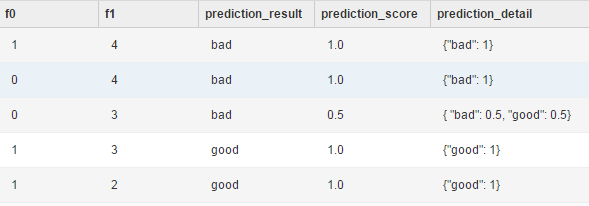## Random forest

A random forest is a classifier that contains multiple decision trees. The output class is the mode of classes of the individual trees.

ID3, C4.5, or CART can be used as the single decision tree algorithm.

#### PAI command

`` PAI -name randomforests ``     -project algo_public``     -DinputTableName="pai_rf_test_input" ``     -DmodelName="pai_rf_test_model" ``     -DforceCategorical="f1" ``     -DlabelColName="class" ``     -DfeatureColNames="f0,f1" ``     -DmaxRecordSize="100000" ``     -DminNumPer="0" ``     -DminNumObj="2" ``     -DtreeNum="3";``

#### Parameter description

Parameter Description Value range Required/Optional, default value/act
inputTableName Input table Table name Required
inputTablePartitions Partitions used for training in the input table, in the format of partition_name=value. The multilevel format is name1=value1/name2=value2. Multiple partitions are separated by commas (,). NA (Optional) All partitions are selected by default.
labelColName Name of the label column in the input table Column name Required
modelName Name of the output model NA Required
treeNum Number of trees in the random forest Positive integer in the range of (0, 1000] Required
weightColName Name of the weight column in the input table NA Optional, default: no weight column
featureColNames Names of feature columns used for training in the input table NA Optional, default: all columns except labelColName and weightColName
excludedColNames Names of feature columns excluded from training in the input table, mutually exclusive with featureColNames NA Optional, default: empty
forceCategorical The default feature parsing rule is: Parse columns of string, boolean, and datetime types as discrete columns, and parse columns of double and bigint types as contiguous columns. You can specify the forceCategorical parameter to parse bigint columns as categorical columns. Optional, default: int columns are parsed as contiguous columns
algorithmTypes Location of the single decision tree algorithm in the forest The value contains two characters. If the forest has n trees and algorithmTypes is set to [a,b], then [0,a) indicates ID3, [a,b) indicates CART, and [b,n) indicates C4.5. If this parameter is set to [2, 4] for a forest with five trees, [0, 1) indicates the ID3 algorithm, [2, 3) indicates the CART algorithm, and 4 indicates the C4.5 algorithm. If the value is None, the algorithms are evenly allocated in the forest. Optional, default: algorithms evenly allocated in the forest
randomColNum Number of random features selected in each split during generation of a single decision tree [1-N], where N is the number of features Optional, default: log2N
minNumObj Minimum number of leaf nodes Positive number Optional, default: 2
minNumPer Minimum ratio of leaf nodes to parent nodes [0,1] Optional, default: 0.0
maxTreeDeep Maximum depth of a tree [1, ∞) Optional, default: ∞
maxRecordSize Number of input random values on each tree in the forest (1000, 1,000,000] Optional, default: 100,000

#### Example

Test data

``create table pai_rf_test_input as``select * from``(``  select 1 as f0,2 as f1, "good" as class from dual``  union all``  select 1 as f0,3 as f1, "good" as class from dual``  union all``  select 1 as f0,4 as f1, "bad" as class from dual``  union all``  select 0 as f0,3 as f1, "good" as class from dual``  union all``  select 0 as f0,4 as f1, "bad" as class from dual``)tmp;``

PAI command

`` PAI -name randomforests ``     -project algo_public``     -DinputTableName="pai_rf_test_input" ``     -Dmodelbashame="pai_rf_test_model" ``     -DforceCategorical="f1" ``     -DlabelColName="class" ``     -DfeatureColNames="f0,f1" ``     -DmaxRecordSize="100000" ``     -DminNumPer="0" ``     -DminNumObj="2" ``     -DtreeNum="3";``

Output description

Model PMML

``<?xml version="1.0" encoding="utf-8"?``<PMML xmlns="http://www.dmg.org/PMML-4_2" xmlns:xsi="http://www.w3.org/2001/XMLSchema-instance" version="4.2" xsi:schemaLocation="http://www.dmg.org/PMML-4_2 http://www.dmg.org/v4-2/pmml-4-2.xsd"``  <Header copyright="Copyright (c) 2014, Alibaba Inc." description=""``    <Application name="ODPS/PMML" version="0.1.0"/``    <TimestampTue, 12 Jul 2016 07:04:48 GMT</Timestamp``  </Header``  <DataDictionary numberOfFields="2"``    <DataField name="f0" optype="continuous" dataType="integer"/``    <DataField name="f1" optype="continuous" dataType="integer"/``    <DataField name="class" optype="categorical" dataType="string"``      <Value value="bad"/``      <Value value="good"/``    </DataField``  </DataDictionary``  <MiningModel modelName="xlab_m_random_forests_1_75078_v0" functionName="classification" algorithmName="RandomForests"``    <MiningSchema``      <MiningField name="f0" usageType="active"/``      <MiningField name="f1" usageType="active"/``      <MiningField name="class" usageType="target"/``    </MiningSchema``    <Segmentation multipleModelMethod="majorityVote"``      <Segment id="0"``        <True/``        <TreeModel modelName="xlab_m_random_forests_1_75078_v0" functionName="classification" algorithmName="RandomForests"``          <MiningSchema``            <MiningField name="f0" usageType="active"/``            <MiningField name="f1" usageType="active"/``            <MiningField name="class" usageType="target"/``          </MiningSchema``          <Node id="1"``            <True/``            <ScoreDistribution value="bad" recordCount="2"/``            <ScoreDistribution value="good" recordCount="3"/``            <Node id="2" score="good"``              <SimplePredicate field="f1" operator="equal" value="2"/``              <ScoreDistribution value="good" recordCount="1"/``            </Node``            <Node id="3" score="good"``              <SimplePredicate field="f1" operator="equal" value="3"/``              <ScoreDistribution value="good" recordCount="2"/``            </Node``            <Node id="4" score="bad"``              <SimplePredicate field="f1" operator="equal" value="4"/``              <ScoreDistribution value="bad" recordCount="2"/``            </Node``          </Node``        </TreeModel``      </Segment``      <Segment id="1"``        <True/``        <TreeModel modelName="xlab_m_random_forests_1_75078_v0" functionName="classification" algorithmName="RandomForests"``          <MiningSchema``            <MiningField name="f0" usageType="active"/``            <MiningField name="f1" usageType="active"/``            <MiningField name="class" usageType="target"/``          </MiningSchema``          <Node id="1"``            <True/``            <ScoreDistribution value="bad" recordCount="2"/``            <ScoreDistribution value="good" recordCount="3"/``            <Node id="2" score="good"``              <SimpleSetPredicate field="f1" booleanOperator="isIn"``                <Array n="2" type="integer"2 3</Array``              </SimpleSetPredicate``              <ScoreDistribution value="good" recordCount="3"/``            </Node``            <Node id="3" score="bad"``              <SimpleSetPredicate field="f1" booleanOperator="isNotIn"``                <Array n="2" type="integer"2 3</Array``              </SimpleSetPredicate``              <ScoreDistribution value="bad" recordCount="2"/``            </Node``          </Node``        </TreeModel``      </Segment``      <Segment id="2"``        <True/``        <TreeModel modelName="xlab_m_random_forests_1_75078_v0" functionName="classification" algorithmName="RandomForests"``          <MiningSchema``            <MiningField name="f0" usageType="active"/``            <MiningField name="f1" usageType="active"/``            <MiningField name="class" usageType="target"/``          </MiningSchema``          <Node id="1"``            <True/``            <ScoreDistribution value="bad" recordCount="2"/``            <ScoreDistribution value="good" recordCount="3"/``            <Node id="2" score="bad"``              <SimplePredicate field="f0" operator="lessOrEqual" value="0.5"/``              <ScoreDistribution value="bad" recordCount="1"/``              <ScoreDistribution value="good" recordCount="1"/``            </Node``            <Node id="3" score="good"``              <SimplePredicate field="f0" operator="greaterThan" value="0.5"/``              <ScoreDistribution value="bad" recordCount="1"/``              <ScoreDistribution value="good" recordCount="2"/``            </Node``          </Node``        </TreeModel``      </Segment``    </Segmentation``  </MiningModel``</PMML``

Model visualization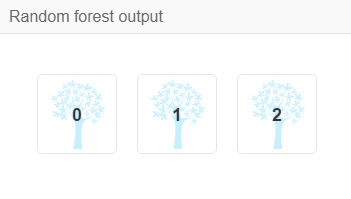## Naive Bayes

Naive Bayes classifier is a simple probabilistic classifier applying Bayes’ theorem with strong (naive) independence assumptions between the features. A probabilistic model that can more accurately describe this potential is called an independent feature model. For details about this algorithm, see Naive Bayes classifier.

Algorithm component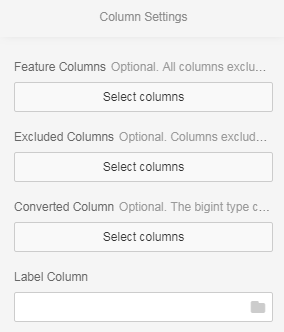• Feature column: Data type of feature columns can be string, double, or bigint.

• Label column: You can select only a column other than feature columns. Its data type can be double, string, or bigint.

#### PAI command

``PAI -name NaiveBayes -project algo_public -DmodelName="xlab_m_NaiveBayes_23772" \``    -DinputTablePartitions="pt=20150501" -DlabelColName="poutcome" \``    -DfeatureColNames="age,previous,cons_conf_idx,euribor3m" \``    -DisFeatureContinuous="1,1,1,1" \``    -DinputTableName="bank_data_partition";``

#### Parameter description

Parameter Description Option Default value
inputTableName (Required) Name of the input table NA NA
inputTablePartitions (Optional) Partitions used for training in the input table Format: Partition_name=value. The multilevel format is name1=value1/name2=value2; multiple partitions are separated by commas All partitions in the input table
modelName (Required) Name of the output model NA NA
labelColName (Required) Name of the label column in the input table NA NA
featureColNames (Optional) Names of feature columns used for training in the input table NA All columns except the label column
excludedColNames Names of feature columns excluded from training in the input table, mutually exclusive with featureColNames NA Empty
forceCategorical (Optional) The default feature parsing rule is: Parse columns of string, boolean, and datetime types as discrete columns, and parse columns of double and bigint types as contiguous columns. You can specify the forceCategorical parameter to parse bigint columns as categorical columns. NA int columns are parsed as contiguous columns

#### Example

Training data

id y f0 f1 f2 f3 f4 f5 f6 f7
1 -1 -0.294118 0.487437 0.180328 -0.292929 -1 0.00149028 -0.53117 -0.0333333
2 +1 -0.882353 -0.145729 0.0819672 -0.414141 -1 -0.207153 -0.766866 -0.666667
3 -1 -0.0588235 0.839196 0.0491803 -1 -1 -0.305514 -0.492741 -0.633333
4 +1 -0.882353 -0.105528 0.0819672 -0.535354 -0.777778 -0.162444 -0.923997 -1
5 -1 -1 0.376884 -0.344262 -0.292929 -0.602837 0.28465 0.887276 -0.6
6 +1 -0.411765 0.165829 0.213115 -1 -1 -0.23696 -0.894962 -0.7
7 -1 -0.647059 -0.21608 -0.180328 -0.353535 -0.791962 -0.0760059 -0.854825 -0.833333
8 +1 0.176471 0.155779 -1 -1 -1 0.052161 -0.952178 -0.733333
9 -1 -0.764706 0.979899 0.147541 -0.0909091 0.283688 -0.0909091 -0.931682 0.0666667
10 -1 -0.0588235 0.256281 0.57377 -1 -1 -1 -0.868488 0.1

Test data

id y f0 f1 f2 f3 f4 f5 f6 f7
1 +1 -0.882353 0.0854271 0.442623 -0.616162 -1 -0.19225 -0.725021 -0.9
2 +1 -0.294118 -0.0351759 -1 -1 -1 -0.293592 -0.904355 -0.766667
3 +1 -0.882353 0.246231 0.213115 -0.272727 -1 -0.171386 -0.981213 -0.7
4 -1 -0.176471 0.507538 0.278689 -0.414141 -0.702128 0.0491804 -0.475662 0.1
5 -1 -0.529412 0.839196 -1 -1 -1 -0.153502 -0.885568 -0.5
6 +1 -0.882353 0.246231 -0.0163934 -0.353535 -1 0.0670641 -0.627669 -1
7 -1 -0.882353 0.819095 0.278689 -0.151515 -0.307329 0.19225 0.00768574 -0.966667
8 +1 -0.882353 -0.0753769 0.0163934 -0.494949 -0.903073 -0.418778 -0.654996 -0.866667
9 +1 -1 0.527638 0.344262 -0.212121 -0.356974 0.23696 -0.836038 -0.8
10 +1 -0.882353 0.115578 0.0163934 -0.737374 -0.56974 -0.28465 -0.948762 -0.933333
1. Create an experiment.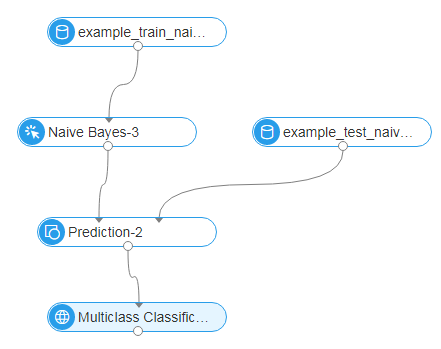2. Select feature columns.3. Select the label column.4. Run the experiment.

• The following model is generated.• The following figure shows the predicted result.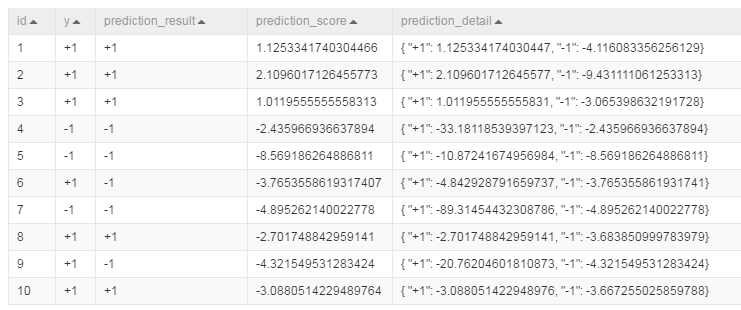## K-means clustering

K-means clustering is the most widely used clustering algorithm, which divides n objects into k clusters to maintain high similarity in each cluster. The similarity is calculated based on the average value of objects in a cluster.

This algorithm randomly selects k objects, each of which originally represents the average value or center of a cluster. Then, the algorithm assigns the remaining objects to the nearest clusters based on their distances from the center of each cluster, and re-calculates the average value of each cluster. This process repeats until the criterion function converges.

The K-means clustering algorithm assumes that object attributes are obtained from the spatial vector, and its objective is to ensure the minimum mean square error sum inside each group.

#### PAI command

``pai -name kmeans``    -project algo_public``    -DinputTableName=pai_kmeans_test_input``    -DselectedColNames=f0,f1``    -DcenterCount=3``    -Dloop=10``    -Daccuracy=0.00001``    -DdistanceType=euclidean``    -DinitCenterMethod=random``    -Dseed=1``    -DmodelName=pai_kmeans_test_input_output_model``    -DidxTableName=pai_kmeans_test_input_output_idx``    -DclusterCountTableName=pai_kmeans_test_input_output_cc``

#### Parameter description

Parameter Description Value range Required/Optional, default value/act
inputTableName Input table Table name Required
selectedColNames Names of the columns used for training in the input table, which are separated by commas, columns of int and double types supported NA Optional, default: all columns in the input table
inputTablePartitions Partitions used for training in the input table, in the format of partition_name=value. The multilevel format is name1=value1/name2=value2. Multiple partitions are separated by commas (,). NA (Optional) All partitions are selected by default.
centerCount Number of clusters Positive integer in the range of [1, 1000] Required
loop Maximum number of iterations Positive integer in the range of [1, 1000] Optional, default: 100
accuracy Algorithm terminal condition. The algorithm is terminated if the variation between two iterations is smaller than this value. NA Optional, default: 0.0
distanceType Distance measuring method euclidean (Euclidean distance), cosine (included angle cosine), cityblock (Manhattan distance) Optional, default: euclidean
initCenterMethod Centroid initialization method random (random sampling), topk (first k rows of the input table), uniform (even distribution), kmpp (k-means++), external (specifying the initial centroid table) Optional, default: random
initCenterTableName Name of the initial centroid table Table name Valid when initCenterMethod is set to external
seed Initial random seed Positive integer Optional, default: current time. If the seed is set to a fixed value, clustering results do not fluctuate greatly.
enableSparse Whether data in the input table is in sparse format true, false Optional, default: false
itemDelimiter Delimiter used between key-value pairs when data in the input table is in sparse format NA Optional, default: space
kvDelimiter Delimiter used between keys and values when data in the input table is in sparse format NA Optional, default: colon
appendColNames Names of appended columns in the inputTableName to be exported to the idxTableName table, which are separated by commas Optional, default: no appended column
modelName Name of the output model Model name Required
idxTableName Clustering result output table corresponding to the input table, specifying class number of each record after clustering Table name Required
idxTablePartition Partition of the output cluster table Table name Optional, default: no partition
clusterCountTableName Output cluster count table, showing the number of nodes in each cluster Optional, no output
centerTableName Output cluster center table NA Optional, about to be deprecated, the modelName parameter recommended

Distance measurement method

Parameter Description
euclideancosine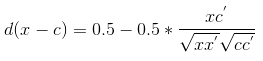cityblockCentroid initialization method

Parameter Description
random Sample K initial centers randomly from the input table. The initial random seed can be specified using the seed parameter.
topk Read the first K rows of the input table as the initial centers
uniform Calculate K evenly distributed initial centers in the input table in ascending order of values
kmpp Use the k-means++ algorithm to select K initial centers. For details about this algorithm, see wiki
external Specify an external initial center table

#### Example

Test data

``create table pai_kmeans_test_input as``select * from``(``  select 1 as f0,2 as f1 from dual``  union all``  select 1 as f0,3 as f1 from dual``  union all``  select 1 as f0,4 as f1 from dual``  union all``  select 0 as f0,3 as f1 from dual``  union all``  select 0 as f0,4 as f1 from dual``)tmp;``

PAI command

``pai -name kmeans``    -project algo_public``    -DinputTableName=pai_kmeans_test_input``    -DselectedColNames=f0,f1``    -DcenterCount=3``    -Dloop=10``    -Daccuracy=0.00001``    -DdistanceType=euclidean``    -DinitCenterMethod=random``    -Dseed=1``    -DmodelName=pai_kmeans_test_input_output_model``    -DidxTableName=pai_kmeans_test_input_output_idx``    -DclusterCountTableName=pai_kmeans_test_input_output_cc``

Output description

Model PMML

``<?xml version="1.0" encoding="utf-8"?``<PMML xmlns="http://www.dmg.org/PMML-4_2" xmlns:xsi="http://www.w3.org/2001/XMLSchema-instance" version="4.2" xsi:schemaLocation="http://www.dmg.org/PMML-4_2 http://www.dmg.org/v4-2/pmml-4-2.xsd"``  <Header copyright="Copyright (c) 2014, Alibaba Inc." description=""``    <Application name="ODPS/PMML" version="0.1.0"/``    <TimestampFri, 15 Jul 2016 03:09:38 GMT</Timestamp``  </Header``  <DataDictionary numberOfFields="2"``    <DataField name="f0" optype="continuous" dataType="integer"/``    <DataField name="f1" optype="continuous" dataType="integer"/``    <DataField name="cluster_index" optype="continuous" dataType="integer"/``  </DataDictionary``  <ClusteringModel modelName="xlab_m_KMeans_2_76889_v0" functionName="clustering" algorithmName="kmeans" modelClass="centerBased" numberOfClusters="3"``    <MiningSchema``      <MiningField name="f0" usageType="active"/``      <MiningField name="f1" usageType="active"/``    </MiningSchema``    <ComparisonMeasure kind="distance" compareFunction="absDiff"``      <squaredEuclidean/``    </ComparisonMeasure``    <ClusteringField field="f0" compareFunction="absDiff"/``    <ClusteringField field="f1" compareFunction="absDiff"/``    <Cluster``      <Array n="2" type="real"0 3.5</Array``    </Cluster``    <Cluster``      <Array n="2" type="real"1 4</Array``    </Cluster``    <Cluster``      <Array n="2" type="real"1 2.5</Array``    </Cluster``  </ClusteringModel``</PMML``

Model visualization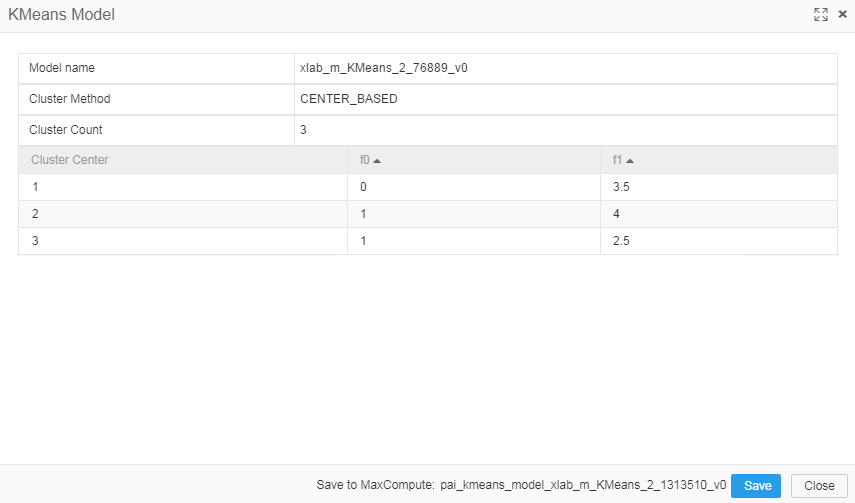Output cluster table: The number of rows equals the total number of rows in the input table. The values in each row represent the cluster numbers of the points in the corresponding row of the input table.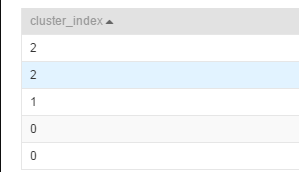Output cluster count table: The number of rows equals the number of clusters. The values in each row represent the number of points in the corresponding clusters.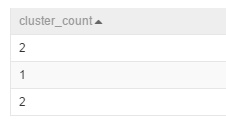## Linear regression

Linear regression is a model used to analyze the linear relationship between a dependent variable and multiple independent variables. For details, see https://en.wikipedia.org/wiki/Linear_regression.

#### PAI command

``PAI -name linearregression ``    -project algo_public ``    -DinputTableName=lm_test_input ``    -DfeatureColNames=x ``    -DlabelColName=y ``    -DmodelName=lm_test_input_model_out;``

#### Algorithm parameters

Parameter Description Option Default value
inputTableName (Required) Name of the input table NA NA
modelName (Required) Name of the output model NA NA
outputTableName (Optional) Name of the output model evaluation table Required when enableFitGoodness is true “”
labelColName (Required) Dependent variable Double or bigint type, only one column allowed NA
featureColNames (Required) Independent variables double or bigint in dense format and string type in sparse format, multiple columns allowed NA
inputTablePartitions (Optional) Partitions in the input table NA “”
maxIter (Optional) Maximum number of iterations NA 100
epsilon (Optional) Minimum likelihood deviation NA 0.000001
enableSparse (Optional) Whether the input table data is in sparse format true, false false
enableFitGoodness (Optional) Whether to perform model evaluation, based on metrics including R-squared, AdjustedR-Squared, AIC, degree of freedom, residual standard deviation, and deviation [true, false] false
enableCoefficientEstimate (Optional) Whether to perform regression coefficient evaluation, based on metrics including value t, value p, and confidence interval [2.5%, 97.5%]. This parameter is valid only when enableFitGoodness is true and is set to false otherwise. true, false false
itemDelimiter (Optional) Delimiter used between key-value pairs in sparse format, valid only when enableSparse is true - Space on the command line interface and comma (,) on web pages
kvDelimiter (Optional) Delimiter used between keys and values in sparse format, valid only when enableSparse is true NA Colon (:)
lifecycle (Optional) Lifecycle of the model evaluation output table 0 -1
coreNum (Optional) Total number of instances [1, 800) Automatically calculated
memSizePerCore (Optional) Memory size for core [1024, 20*1024] Automatically calculated

#### Example

Test data

SQL statement for data generation:

``  drop table if exists lm_test_input;``  create table lm_test_input as ``  select ``    *``  from ``  (``    select 10 as y, 1.84 as x1, 1 as x2, '0:1.84 1:1' as sparsecol1 from dual``      union all``    select 20 as y, 2.13 as x1, 0 as x2, '0:2.13' as sparsecol1 from dual``      union all``    select 30 as y, 3.89 as x1, 0 as x2, '0:3.89' as sparsecol1 from dual``      union all``    select 40 as y, 4.19 as x1, 0 as x2, '0:4.19' as sparsecol1 from dual``      union all``    select 50 as y, 5.76 as x1, 0 as x2, '0:5.76' as sparsecol1 from dual``      union all``    select 60 as y, 6.68 as x1, 2 as x2, '0:6.68 1:2' as sparsecol1 from dual``      union all``    select 70 as y, 7.58 as x1, 0 as x2, '0:7.58' as sparsecol1 from dual``      union all``    select 80 as y, 8.01 as x1, 0 as x2, '0:8.01' as sparsecol1 from dual``      union all``    select 90 as y, 9.02 as x1, 3 as x2, '0:9.02 1:3' as sparsecol1 from dual``      union all``    select 100 as y, 10.56 as x1, 0 as x2, '0:10.56' as sparsecol1 from dual``  ) tmp;``

Running command

``PAI -name linearregression ``    -project algo_public ``    -DinputTableName=lm_test_input ``    -DlabelColName=y ``    -DfeatureColNames=x1,x2 ``    -DmodelName=lm_test_input_model_out ``    -DoutputTableName=lm_test_input_conf_out ``    -DenableCoefficientEstimate=true ``    -DenableFitGoodness=true ``    -Dlifecycle=1;``PAI -name prediction ``    -project algo_public ``    -DmodelName=lm_test_input_model_out``    -DinputTableName=lm_test_input ``    -DoutputTableName=lm_test_input_predict_out ``    -DappendColNames=y;``

Running result

lm_test_input_conf_out

``+------------+------------+------------+------------+--------------------+------------+``| colname    | value      | tscore     | pvalue     | confidenceinterval | p          |``+------------+------------+------------+------------+--------------------+------------+``| Intercept  | -6.42378496687763 | -2.2725755951390028 | 0.06       | {"2.5%": -11.964027, "97.5%": -0.883543} | coefficient |``| x1         | 10.260063429838898 | 23.270944360826963 | 0.0        | {"2.5%": 9.395908, "97.5%": 11.124219} | coefficient |``| x2         | 0.35374498323846265 | 0.2949247320997519 | 0.81       | {"2.5%": -1.997160, "97.5%": 2.704650} | coefficient |``| rsquared   | 0.9879675667384592 | NULL       | NULL       | NULL               | goodness   |``| adjusted_rsquared | 0.9845297286637332 | NULL       | NULL       | NULL               | goodness   |``| aic        | 59.331109494251805 | NULL       | NULL       | NULL               | goodness   |``| degree_of_freedom | 7.0        | NULL       | NULL       | NULL               | goodness   |``| standardErr_residual | 3.765777749448906 | NULL       | NULL       | NULL               | goodness   |``| deviance   | 99.26757440771128 | NULL       | NULL       | NULL               | goodness   |``+------------+------------+------------+------------+--------------------+------------+``

lm_test_input_predict_out

``+------------+-------------------+------------------+-------------------+``| y          | prediction_result | prediction_score | prediction_detail |``+------------+-------------------+------------------+-------------------+``| 10         | NULL              | 12.808476727264404 | {"y": 12.8084767272644} |``| 20         | NULL              | 15.43015013867922 | {"y": 15.43015013867922} |``| 30         | NULL              | 33.48786177519568 | {"y": 33.48786177519568} |``| 40         | NULL              | 36.565880804147355 | {"y": 36.56588080414735} |``| 50         | NULL              | 52.674180388994415 | {"y": 52.67418038899442} |``| 60         | NULL              | 62.82092871092313 | {"y": 62.82092871092313} |``| 70         | NULL              | 71.34749583130122 | {"y": 71.34749583130122} |``| 80         | NULL              | 75.75932310613193 | {"y": 75.75932310613193} |``| 90         | NULL              | 87.1832221199846 | {"y": 87.18322211998461} |``| 100        | NULL              | 101.92248485222113 | {"y": 101.9224848522211} |``+------------+-------------------+------------------+-------------------+``

## GBDT regression

GBDT, short for gradient boosting decision tree, is an iterative decision tree algorithm based on multiple decision trees. The final output is the sum of conclusions of all trees. GBDT applies to almost all regression models (linear or nonlinear) and has a wider scope of application than logistic regression that only applies to linear regression. For details, see the following references (a) A Regression Framework for Learning Ranking Functions Using Relative Relevance Judgments, (b) From RankNet to LambdaRank to LambdaMART: An Overview.

#### PAI command

``PAI -name gbdt``    -project algo_public``    -DfeatureSplitValueMaxSize="500"``    -DlossType="0"``    -DrandSeed="0"``    -DnewtonStep="0"``    -Dshrinkage="0.05"``    -DmaxLeafCount="32"``    -DlabelColName="campaign"``    -DinputTableName="bank_data_partition"``    -DminLeafSampleCount="500"``    -DsampleRatio="0.6"``    -DgroupIDColName="age"``    -DmaxDepth="11"``    -DmodelName="xlab_m_GBDT_83602"``    -DmetricType="2"``    -DfeatureRatio="0.6"``    -DinputTablePartitions="pt=20150501"``    -Dtau="0.6"``    -Dp="1"``    -DtestRatio="0.0"``    -DfeatureColNames="previous,cons_conf_idx,euribor3m"``    -DtreeCount="500"``

#### Parameter description

Parameter Description Value range Required/Optional, default value
inputTableName Input table Table name Required
featureColNames Names of the feature columns used for training in the input table Column names Optional, default: all columns with values
labelColName Name of the label column in the input table Column name Required
inputTablePartitions Partitions used for training in the input table, in the format of partition_name=value. The multilevel format is name1=value1/name2=value2. Multiple partitions are separated by commas (,). NA (Optional) All partitions are selected by default.
modelName Name of the output model NA Required
outputImportanceTableName Name of the output feature importance table NA Optional
groupIDColName Name of a grouping column Column name Optional, default: full table
lossType Loss function type, 0: GBRANK, 1: LAMBDAMART_DCG, 2: LAMBDAMART_NDCG, 3: LEAST_SQUARE, 4: LOG_LIKELIHOOD 0, 1, 2, 3, 4 Optional, default: 0
metricType Metric type, 0(NDCG)-: normalized discounted cumulative gain; 1(DCG): discounted cumulative gain; 2 (AUC) adaptive only to 0/1 label 0, 1, 2 Optional, default: 2
treeCount Number of trees [1, 10,000] Optional, default: 500
shrinkage Learning rate (0, 1] Optional, default: 0.05
maxLeafCount Maximum number of leaves, which must be an integer [2, 1000] Optional, default: 32
maxDepth Maximum depth of a tree, which must be an integer [1, 11] Optional, default: 11
minLeafSampleCount Minimum number of samples on a leaf node, which must be an integer [100, 1000] Optional, default: 500
sampleRatio Ratio of samples collected during the training (0, 1] Optional, default: 0.6
featureRatio Ratio of features collected during the training (0, 1] Optional, default: 0.6
tau Parameter Tau in gbrank loss [0, 1] Optional, default: 0.6
p Parameter p in gbrank loss [1, 10] Optional, default: 1
randSeed Random seed [0, 10] Optional, default: 0
newtonStep Whether to use the newton method 0,1 Optional, default: 1
featureSplitValueMaxSize Number of records that a feature can split into [1, 1000] Optional, default: 500
lifecycle Lifecycle of the output table NA Optional, default: not set

#### Example

Data generation

``drop table if exists gbdt_ls_test_input;``create table gbdt_ls_test_input``as``select``    *``from``(``    select``        cast(1 as double) as f0,``        cast(0 as double) as f1,``        cast(0 as double) as f2,``        cast(0 as double) as f3,``        cast(0 as bigint) as label``    from dual``    union all``        select``            cast(0 as double) as f0,``            cast(1 as double) as f1,``            cast(0 as double) as f2,``            cast(0 as double) as f3,``            cast(0 as bigint) as label``    from dual``    union all``        select``            cast(0 as double) as f0,``            cast(0 as double) as f1,``            cast(1 as double) as f2,``            cast(0 as double) as f3,``            cast(1 as bigint) as label``    from dual``    union all``        select``            cast(0 as double) as f0,``            cast(0 as double) as f1,``            cast(0 as double) as f2,``            cast(1 as double) as f3,``            cast(1 as bigint) as label``    from dual``    union all``        select``            cast(1 as double) as f0,``            cast(0 as double) as f1,``            cast(0 as double) as f2,``            cast(0 as double) as f3,``            cast(0 as bigint) as label``    from dual``    union all``        select``            cast(0 as double) as f0,``            cast(1 as double) as f1,``            cast(0 as double) as f2,``            cast(0 as double) as f3,``            cast(0 as bigint) as label``    from dual``) a;``

PAI command

Training

``drop offlinemodel if exists gbdt_ls_test_model;``PAI -name gbdt``    -project algo_public``    -DfeatureSplitValueMaxSize="500"``    -DlossType="3"``    -DrandSeed="0"``    -DnewtonStep="1"``    -Dshrinkage="0.5"``    -DmaxLeafCount="32"``    -DlabelColName="label"``    -DinputTableName="gbdt_ls_test_input"``    -DminLeafSampleCount="1"``    -DsampleRatio="1"``    -DmaxDepth="10"``    -DmetricType="0"``    -DmodelName="gbdt_ls_test_model"``    -DfeatureRatio="1"``    -Dp="1"``    -Dtau="0.6"``    -DtestRatio="0"``    -DfeatureColNames="f0,f1,f2,f3"``    -DtreeCount="10"``

Prediction

``drop table if exists gbdt_ls_test_prediction_result;``PAI -name prediction``    -project algo_public``    -DdetailColName="prediction_detail"``    -DmodelName="gbdt_ls_test_model"``    -DitemDelimiter=","``    -DresultColName="prediction_result"``    -Dlifecycle="28"``    -DoutputTableName="gbdt_ls_test_prediction_result"``    -DscoreColName="prediction_score"``    -DkvDelimiter=":"``    -DinputTableName="gbdt_ls_test_input"``    -DenableSparse="false"``    -DappendColNames="label"``

Input description

gbdt_ls_test_input

f0 f1 f2 f3 label
1.0 0.0 0.0 0.0 0
0.0 0.0 1.0 0.0 1
0.0 0.0 0.0 1.0 1
0.0 1.0 0.0 0.0 0
1.0 0.0 0.0 0.0 0
0.0 1.0 0.0 0.0 0

Output description

gbdt_ls_test_prediction_result

label prediction_result prediction_score prediction_detail
0 NULL 0.0 {“label”: 0}
0 NULL 0.0 {“label”: 0}
1 NULL 0.9990234375 {“label”: 0.9990234375}
1 NULL 0.9990234375 {“label”: 0.9990234375}
0 NULL 0.0 {“label”: 0}
0 NULL 0.0 {“label”: 0}

## Collaborative filtering (etrec)

• Etrec is an item-based collaborative filtering algorithm that uses two input columns and provides the top K items with the highest similarity as the output.

#### PAI command

``PAI -name pai_etrec``    -project algo_public``    -DsimilarityType="wbcosine"``    -Dweight="1"``    -DminUserBehavior="2"``    -Dlifecycle="28"``    -DtopN="2000"``    -Dalpha="0.5"``    -DoutputTableName="etrec_test_result"``    -DmaxUserBehavior="500"``    -DinputTableName="etrec_test_input"``    -Doperator="add"``    -DuserColName="user"``    -DitemColName="item"``

#### Parameter description

Parameter Description Option Default value
inputTableName (Required) Name of the input table NA NA
userColName (Required) Name of the selected user column in the input table NA NA
itemColName (Required) Name of the selected item column in the input table NA NA
inputTablePartitions (Optional) Names of the selected partitions in the input table NA All partitions in the input table
outputTableName (Required) Name of the output table NA NA
outputTablePartition (Optional) Partition of the output table NA No partition
similarityType (Optional) Type of similarity wbcosine, asymcosine, jaccard wbcosine
topN (Optional) N items with the highest similarity [1, 10,000] 2000
minUserBehavior (Optional) Minimum user behavior [2,) 2
maxUserBehavior (Optional) Maximum user behavior [2, 100,000] 500
itemDelimiter (Optional) Delimiter used between items in the output table NA “ “
kvDelimiter (Optional) Delimiter used between keys and values in the output table NA “:”
alpha (Optional) Value of the smoothing factor for asymcosine NA 0.5
weight (Optional) Weighting exponent for asymcosine NA 1.0
operator (Optional) Action taken when the same items exist for one user add, mul, min, max add
lifecycle (Optional) Lifecycle of the output table NA 1

#### Example

Data generation

``drop table if exists etrec_test_input;``create table etrec_test_input``as``select``    *``from``(``    select``        cast(0 as string) as user,``        cast(0 as string) as item``    from dual``    union all``        select``            cast(0 as string) as user,``            cast(1 as string) as item``        from dual``    union all``        select``            cast(1 as string) as user,``            cast(0 as string) as item``        from dual``    union all``        select``            cast(1 as string) as user,``            cast(1 as string) as item``        from dual``) a;``

PAI command

``drop table if exists etrec_test_result;``PAI -name pai_etrec``    -project algo_public``    -DsimilarityType="wbcosine"``    -Dweight="1"``    -DminUserBehavior="2"``    -Dlifecycle="28"``    -DtopN="2000"``    -Dalpha="0.5"``    -DoutputTableName="etrec_test_result"``    -DmaxUserBehavior="500"``    -DinputTableName="etrec_test_input"``    -Doperator="add"``    -DuserColName="user"``    -DitemColName="item";``

Input descriptionetrec_test_input

user item
0 0
0 1
1 0
1 1

Output descriptionetrec_test_result

itemid similarity
0 1:1
1 0:1

## Confusion matrix

The confusion matrix is a visualization tool typically used in supervised learning. (In unsupervised learning, it is called a matching matrix). This tool is used to compare classification results with actual measured values and display the accuracy of classification results in a confusion matrix. For more information about the confusion matrix, see Confusion matrix.

Component connection: Generally, the classification prediction component is on the upper layer. The following figure shows an example.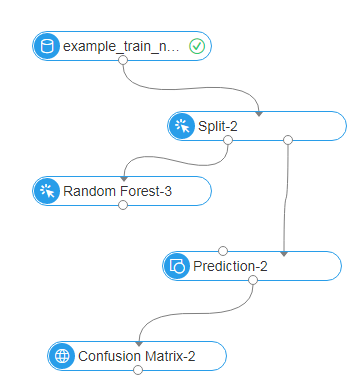#### Parameter settings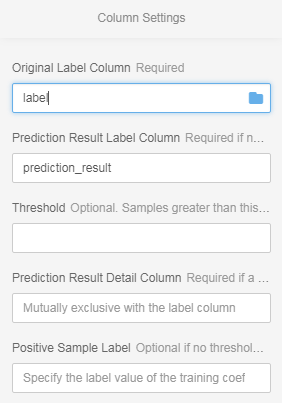NOTE: Only one of the label column and detail column can be selected in the predicted result table.

#### Evaluation report

Right-click the confusion matrix to view the evaluation report.

• Confusion matrix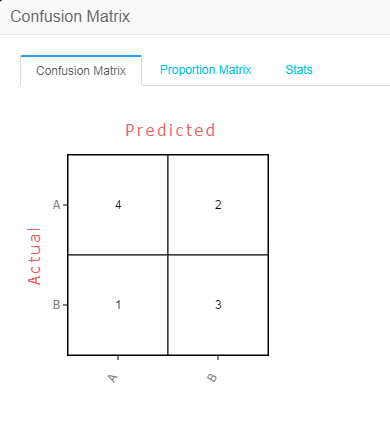• The following figure shows information about each label, including the metrics, accuracy, and recall rate.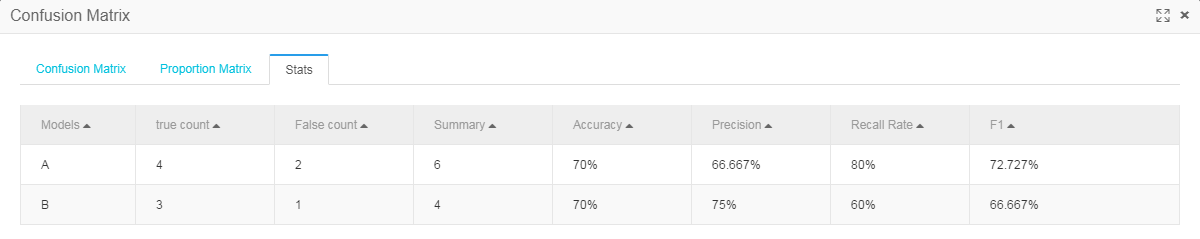#### PAI command

• Sample of a PAI command without a threshold specified
``pai -name confusionmatrix -project algo_public \``    -DinputTableName=wpbc_pred \``    -DlabelColName=label \``    -DpredictionColName=prediction_result \``    -DoutputTableName=wpbc_confu;``
• Sample of a PAI command with a threshold specified
``pai -name confusionmatrix -project algo_public \``    -DinputTableName=wpbc_pred \``    -DlabelColName=label \``    -DpredictionDetailColName=prediction_detail \``    -DgoodValue=N \``    -Dthreshold=0.8 \``    -DoutputTableName=wpbc_confu;``

#### Parameter description

Parameter key Description Option Default value
inputTableName (Required) Name of the input table (prediction output table) NA NA
labelColName (Required) Name of the original label column NA NA
outputTableName (Required) Name of the output table that stores the confusion matrix NA NA
inputTablePartition (Optional) Partition of the input table NA All partitions in the input table
predictionColName (Optional) Name of the label column of the predicted result table, required when no threshold is specified NA NA
predictionDetailColName (Optional) Name of the detail column of the predicted result table, required when a threshold is specified NA NA
threshold (Optional) Threshold for a good value NA 0.5
goodValue (Optional) Label value of a training coefficient during binary classification, required when a threshold is specified NA NA
lifecycle (Optional) Lifecycle of the output table Positive integer No lifecycle

#### Example

Test data

id label prediction_result
0 A A
1 A B
2 A A
3 A A
4 B B
5 B B
6 B A
7 B B
8 B A
9 A A
1. Create an experiment.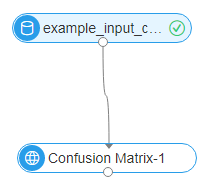2. Configuration parameters.3. Run the experiment.

• The confusion matrix is as follows:• The following figure shows statistics about each label.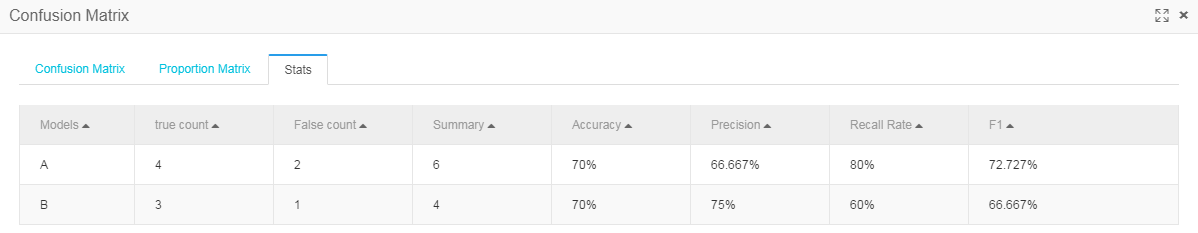## Multiclass classification evaluation

This component evaluates a multiclass classification algorithm model based on the accuracy, kappa, F1 score, and other metrics in the predicted result and expected result of the classification model.

Component connection: The multiclass classification evaluation component needs to be connected to the prediction component and does not support regression models. The following figure shows an example of connection between components.#### Parameter settings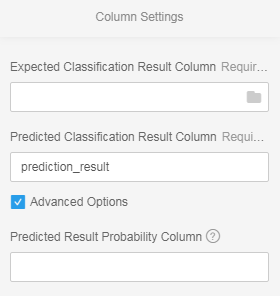• In the Expected Classification Result Column drop-down list, you can select the Original Label column.

NOTE: A maximum of 1000 classes are supported.

• In the Predicted Classification Result Column drop-down list, you can select the Predicted Label column. The default value is prediction_result.

• In the Predicted Result Probability Column drop-down list, you can select the Predicted Label Probability column. Generally, the name of this field is prediction_detail.

NOTE: This parameter applies only to random forest prediction.

#### Evaluation report description

• Metrics of each label are calculated using the one-vs-all method, as shown in the following figure.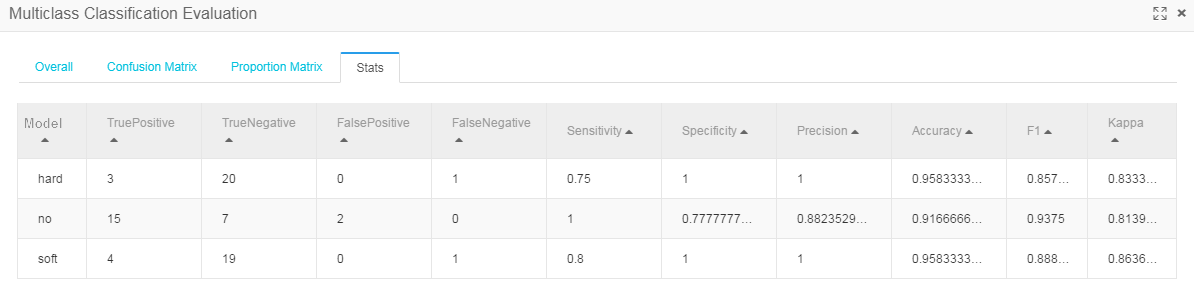• The following figure shows the summary of metrics, among which MacroAveraged indicates the average value of each label’s metrics.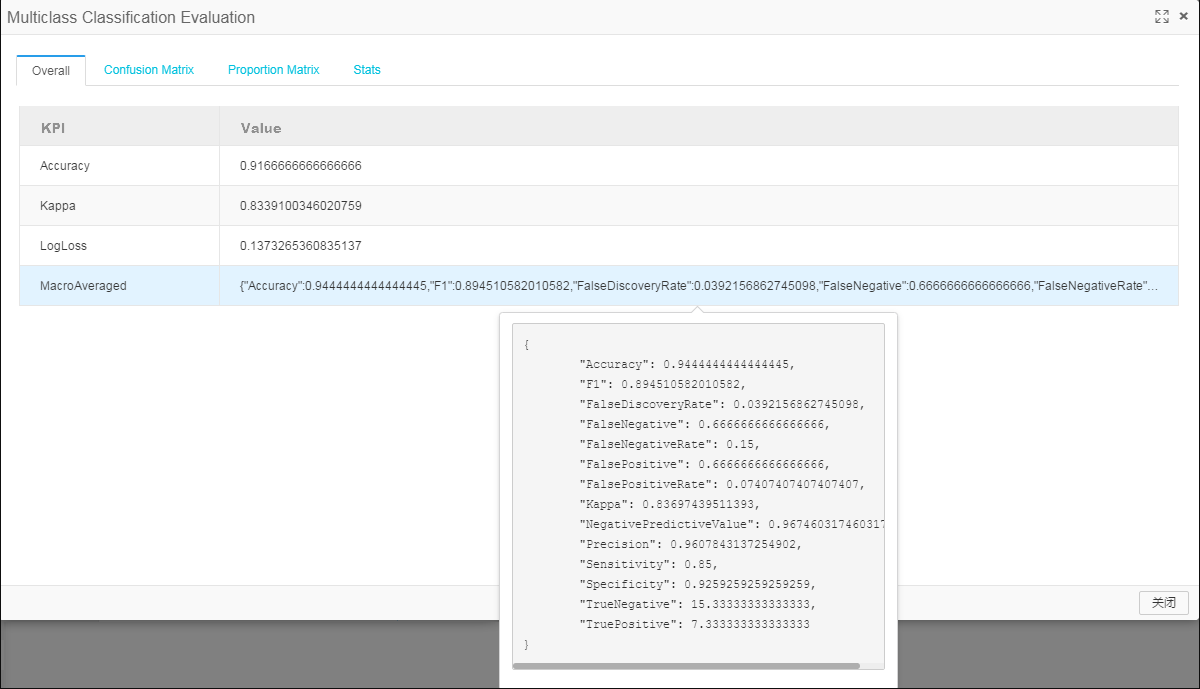• Confusion matrix.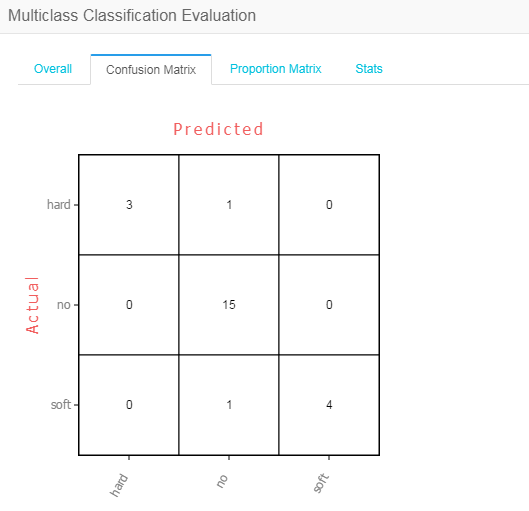#### PAI command

``PAI -name MultiClassEvaluation -project algo_public \``    –DinputTableName="test_input" \``    -DoutputTableName="test_output" \``    -DlabelColName="label" \``    -DpredictionColName="prediction_result" \``    -Dlifecycle=30;``

#### Parameter settings

Parameter Description Option Default value
inputTableName (Required) Name of the input table NA NA
inputTablePartitions (Optional) Partitions used for calculation in the input table NA All partitions in the input table
outputTableName (Required) Name of the output table NA NA
labelColName (Required) Name of the original label column in the input table NA NA
predictionColName (Required) Name of the label column of the predicted result table NA NA
predictionDetailColName (Optional) Name of the predicted result probability column, in the format of {“A“:0.2, “B“:0.3, “C“, 0.5} NA Empty
lifecycle (Optional) Lifecycle of the output table Positive integer No lifecycle
coreNum (Optional) Number of cores for calculation Positive integer Automatically assigned
memSizePerCore (Optional) Memory size for each core, in MB Positive integer in the range of (0, 65536) Automatically assigned

#### Description of the output table in JSON format

``{``    "LabelNumber": 3,``    "LabelList": ["A", "B", "C"],``    "ConfusionMatrix": [ // Confusion matrix [actual][predict]``        [100, 10, 20],``        [30, 50, 9],``        [7, 40, 90] ],``    "ProportionMatrix": [ // Proportion in each row [actual][predict]``        [0.6, 0.2, 0.2],``        [0.3, 0.6, 0.1],``        [0.1, 0.4, 0.5] ],``    "ActualLabelFrequencyList": [ // Actual frequency of each label``        200, 300, 600],``    "ActualLabelProportionList": [ // Actual proportion of each label``        0.1, 0.2, 0.7],``    "PredictedLabelFrequencyList": [ // Predicted frequency of each label``        300, 400, 400],``    "PredictedLabelProportionList": [ // Predicted proportion of each label``        0.2, 0.1, 0.7],``    "OverallMeasures": {        // Overall metrics``        "Accuracy": 0.70,``        "Kappa" : 0.3,``        "MacroList": {       // Average value of each label's metrics``            "Sensitivity": 0.4,``            "Specificity": 0.3,``        },``        "MicroList": {      // Metrics calculated based on the sum of TP, TN, FP, and FN values of each label``            "Sensitivity": 0.4,``            "Specificity": 0.3,``        },``        "LabelFrequencyBasedMicro": { // Frequency-based weighted average value of each label's metrics``            "Sensitivity": 0.4,``            "Specificity": 0.3,``        },``    },``    "LabelMeasuresList": [      // Metrics of each label``        {``            "Accuracy": 0.6,``            "Sensitivity": 0.4,``            "Specificity": 0.3,``            "Kappa": 0.3``        },``        {``            "Accuracy": 0.6,``            "Sensitivity": 0.4,``            "Specificity": 0.3,``            "Kappa": 0.3``        },``    ]``}``

#### Example

Test data

data table example_input_mc_eval

id label prediction detail
0 A A {“A”: 0.6, “B”: 0.4}
1 A B {“A”: 0.45, “B”: 0.55}
2 A A {“A”: 0.7, “B”: 0.3}
3 A A {“A”: 0.9, “B”: 0.1}
4 B B {“A”: 0.2, “B”: 0.8}
5 B B {“A”: 0.1, “B”: 0.9}
6 B A {“A”: 0.52, “B”: 0.48}
7 B B {“A”: 0.4, “B”: 0.6}
8 B A {“A”: 0.6, “B”: 0.4}
9 A A {“A”: 0.75, “B”: 0.25}
1. Create an experiment.2. Configuration parameters.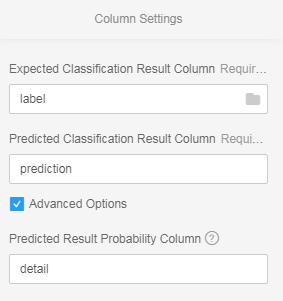3. Run the experiment.

• The overall report is as follows:• Statistics about each label are as follows:• The output table in JSON format is as follows:

``{``    "ActualLabelFrequencyList": [5,``        5],``    "ActualLabelProportionList": [0.5,``        0.5],``    "ConfusionMatrix": [[4,``            1],``        [2,``            3]],``    "LabelList": ["A",``        "B"],``    "LabelMeasureList": [{``            "Accuracy": 0.7,``            "Auc": 0.9,``            "F1": 0.7272727272727273,``            "FalseDiscoveryRate": 0.3333333333333333,``            "FalseNegative": 1,``            "FalseNegativeRate": 0.2,``            "FalsePositive": 2,``            "FalsePositiveRate": 0.4,``            "Kappa": 0.3999999999999999,``            "NegativePredictiveValue": 0.75,``            "Precision": 0.6666666666666666,``            "Sensitivity": 0.8,``            "Specificity": 0.6,``            "TrueNegative": 3,``            "TruePositive": 4},``        {``            "Accuracy": 0.7,``            "Auc": 0.9,``            "F1": 0.6666666666666666,``            "FalseDiscoveryRate": 0.25,``            "FalseNegative": 2,``            "FalseNegativeRate": 0.4,``            "FalsePositive": 1,``            "FalsePositiveRate": 0.2,``            "Kappa": 0.3999999999999999,``            "NegativePredictiveValue": 0.6666666666666666,``            "Precision": 0.75,``            "Sensitivity": 0.6,``            "Specificity": 0.8,``            "TrueNegative": 4,``            "TruePositive": 3}],``    "LabelNumber": 2,``    "OverallMeasures": {``        "Accuracy": 0.7,``        "Kappa": 0.3999999999999999,``        "LabelFrequencyBasedMicro": {``            "Accuracy": 0.7,``            "F1": 0.696969696969697,``            "FalseDiscoveryRate": 0.2916666666666666,``            "FalseNegative": 1.5,``            "FalseNegativeRate": 0.3,``            "FalsePositive": 1.5,``            "FalsePositiveRate": 0.3,``            "Kappa": 0.3999999999999999,``            "NegativePredictiveValue": 0.7083333333333333,``            "Precision": 0.7083333333333333,``            "Sensitivity": 0.7,``            "Specificity": 0.7,``            "TrueNegative": 3.5,``            "TruePositive": 3.5},``        "LogLoss": 0.4548640449724484,``        "MacroAveraged": {``            "Accuracy": 0.7,``            "F1": 0.696969696969697,``            "FalseDiscoveryRate": 0.2916666666666666,``            "FalseNegative": 1.5,``            "FalseNegativeRate": 0.3,``            "FalsePositive": 1.5,``            "FalsePositiveRate": 0.3,``            "Kappa": 0.3999999999999999,``            "NegativePredictiveValue": 0.7083333333333333,``            "Precision": 0.7083333333333333,``            "Sensitivity": 0.7,``            "Specificity": 0.7,``            "TrueNegative": 3.5,``            "TruePositive": 3.5},``        "MicroAveraged": {``            "Accuracy": 0.7,``            "F1": 0.7,``            "FalseDiscoveryRate": 0.3,``            "FalseNegative": 3,``            "FalseNegativeRate": 0.3,``            "FalsePositive": 3,``            "FalsePositiveRate": 0.3,``            "Kappa": 0.3999999999999999,``            "NegativePredictiveValue": 0.7,``            "Precision": 0.7,``            "Sensitivity": 0.7,``            "Specificity": 0.7,``            "TrueNegative": 7,``            "TruePositive": 7}},``    "PredictedLabelFrequencyList": [6,``        4],``    "PredictedLabelProportionList": [0.6,``        0.4],``    "ProportionMatrix": [[0.8,``            0.2],``        [0.4,``            0.6]]}``

## Binary classification evaluation

The evaluation module can calculate the AUC, KS, and F1 score, and provide output data for drawing the KS curve, PR curve, ROC curve, LIFT chart, and gain chart. The module also supports grouping evaluation.

#### PAI command

``pai -name=evaluate -project=algo_public``    -DoutputMetricTableName=output_metric_table``    -DoutputDetailTableName=output_detail_table``    -DinputTableName=input_data_table``    -DlabelColName=label``    -DscoreColName=score``

#### Parameter description

Parameter Description Option Default value
inputTableName (Required) Name of the input table NA NA
inputTablePartitions (Optional) Partitions in the input table - All partitions in the input table
labelColName (Required) Name of the label column NA NA
scoreColName (Required) Name of the score column NA NA
groupColName (Optional) Name of the group column used in grouping evaluation. NA NA
binCount (Optional) Number of equal-frequency bins used to calculate metrics such as KS and PR NA 1000
outputMetricTableName (Required) Name of the output metric table, consisting of two columns (Metric and Value) and three rows (AUC, KS, and F1 Score) NA NA
outputDetailTableName (Optional) Name of the output detail table used for chart drawing NA NA
positiveLabel (Optional) Class of the positive sample NA 1
lifecycle (Optional) Life cycle of the output table NA Unspecified by default
coreNum (Optional) Number of cores NA Automatically calculated by default
memSizePerCore (Optional) Size of memory NA Automatically calculated by default

#### Parameter settings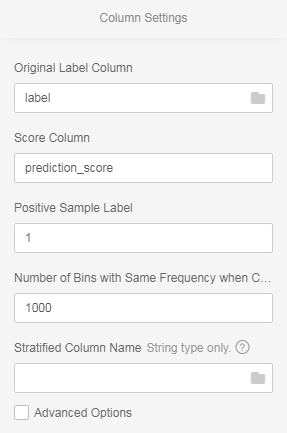• In the Original Label Column drop-down list, you can select the Original Label column.
• In the Score Column box, you can select the Prediction Score column. The default option is prediction_score.
• In the Positive Sample Label box, you can select the label value corresponding to the positive sample.
• In the Number of Bins with Same Frequency when Calculating Indexes such as KS and PR box, you can determine how many equal-frequency bins to divide data into. The default value is 1000.
• In the Grouping Columns box, you can select the grouping columns used to divide data during grouping evaluation.

#### Evaluation result display

Right-click the Binary Classification Evaluation node and select View Evaluation Report from the shortcut menu to view the evaluation result, as shown in the following figure.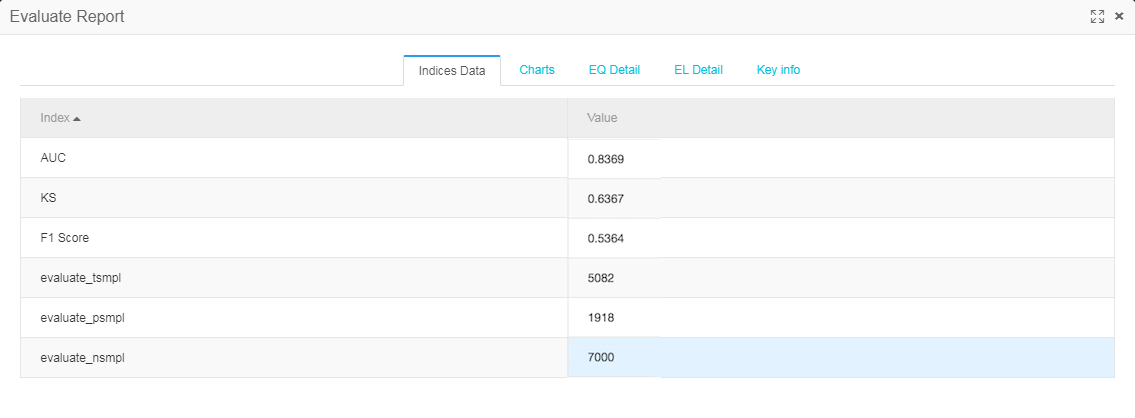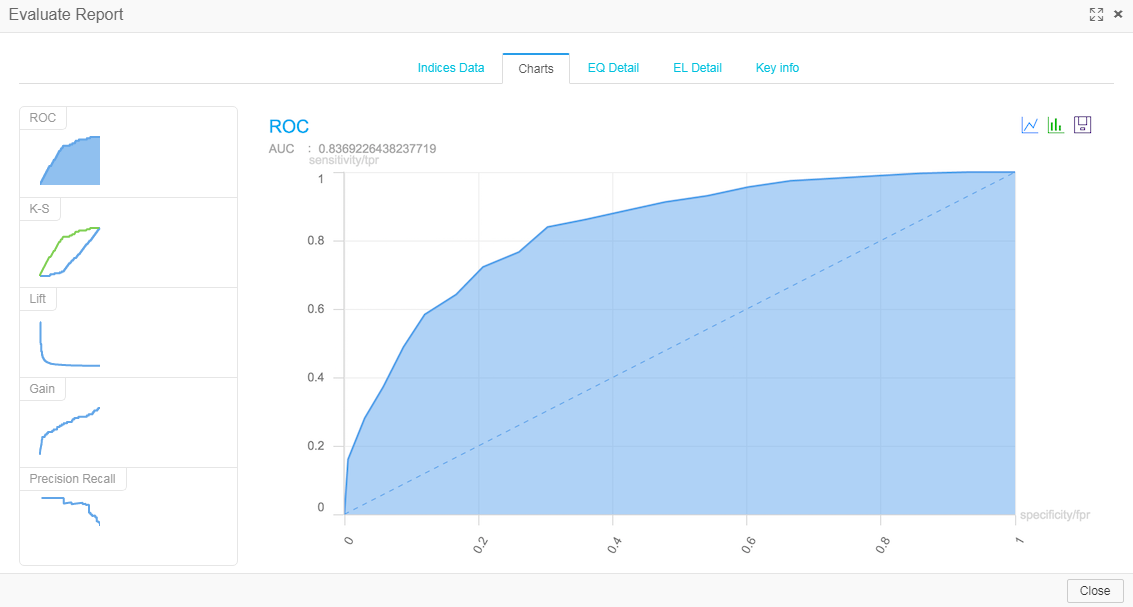## Regression model evaluation

This component evaluates a regression algorithm model based on metrics and residual histograms in the predicted result and expected result. The metrics include SST, SSE, SSR, R2, R, MSE, RMSE, MAE, MAD, MAPE, count, yMean, and predictMean.

#### PAI command

``PAI -name regression_evaluation -project algo_public ``    -DinputTableName=input_table ``    -DyColName=y_col ``    -DpredictionColName=prediction_col ``    -DindexOutputTableName=index_output_table``    -DresidualOutputTableName=residual_output_table;``
Parameter Description Option Default value
inputTableName (Required) Name of the input table NA NA
inputTablePartitions (Optional) Partitions used for calculation in the input table NA All partitions in the input table
yColName (Required) Name of the original dependent variable column in the input table, numerical value NA NA
predictionColName (Required) Name of the predicted dependent variable column, numerical value NA NA
indexOutputTableName (Required) Name of the regression metric output table NA NA
residualOutputTableName (Required) Name of the residual histogram output table NA NA
intervalNum (Optional) Number of intervals in a histogram NA 100
lifecycle (Optional) Lifecycle of the output table Positive integer No lifecycle
coreNum (Optional) Number of instances NA Not set
memSizePerCore (Optional) Memory size for each core NA Not set

#### Output result

##### Regression metric output table

The output table is in JSON format. JSON fields are described as follows:

Field Description
SST Total sum of squares
SSE Sum of squared errors
SSR Sum of squares due to regression
R2 Coefficient of determination
R Coefficient of multiple correlation
MSE Mean squared error
RMSE Root-mean-square error
MAE Mean absolute error
MAPE Mean absolute percentage error
count Number of rows
yMean Mean of original dependent variables
predictionMean Mean of predicted results

## Prediction

The prediction component is used to make model-based predictions. The component has two inputs (training model and prediction data) and one output (predicted result).
Traditional data mining algorithms all use this component to make predictions.

#### PAI command

``PAI -name prediction``    -DmodelName=nb_model``    -DinputTableName=wpbc``    -DoutputTableName=wpbc_pred``    -DappendColNames=label;``

#### Parameter description

Parameter Description Value range Required/Optional, default value/act
inputTableName Name of the input table Table name Required
modelName Name of a model Model name Required
outputTableName Name of the output table Table name Required
featureColNames Names of the feature columns used for prediction in the input table Column names Optional, default: all columns
appendColNames Names of the columns in the prediction input table to be appended to the output table Optional, default: no appended column
inputTablePartitions Partitions used for prediction in the input table, in the format of partition_name=value. The multilevel format is name1=value1/name2=value2; multiple partitions are separated by commas NA Optional, default: all partitions in the input table
outputTablePartition Partition in the output table NA Optional, default: no partition
resultColName Name of the result column in the output table NA Optional, default: prediction_result
scoreColName Name of the score column in the output table NA Optional, default: prediction_score
detailColName Name of the detail column in the output table NA Optional, default: prediction_detail
enableSparse Whether data in the input table is in sparse format true, false Optional, default: false
itemDelimiter Delimiter used between key-value pairs when data in the input table is in sparse format NA Optional, default: space
kvDelimiter Delimiter used between keys and values when data in the input table is in sparse format NA Optional, default: colon
lifecycle Lifecycle of the output table Positive integer Optional, default: no lifecycle

#### Prediction formula

##### Naive Bayes

Prediction_result formula: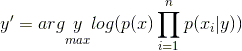Prediction_score formula: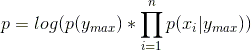Classification variables: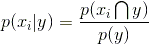Continuous variables: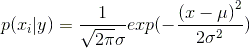#### K-means

Prediction_result formula: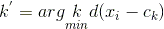Prediction_score formulas:

euclidean:cosine:cityblock:#### Output description

Classification Model Prediction_result Prediction_score Prediction_detail
Binary classification Logistic regression model Predicted label Predicted label probability Each label and its probability
Linear SVM model Predicted label Predicted label probability Each label and its probability
Random forest model Predicted label Predicted label probability Each label and its probability
GBDT_LR model Predicted label Predicted label probability Each label and its probability
Naive Bayes model Predicted label Log (predicted label probability) Each label and its log (probability)
XGboost model Predicted label Predicted label probability Each label and its probability
Multiclass classification Logistic regression model Predicted label Predicted label probability Each label and its probability
Random forest model Predicted label Predicted label probability Label of each leaf node and its probability
Naive Bayes model Predicted label Log (predicted label probability) Each label and its log (probability)
Regression Linear regression model Empty Regression value Label column name: regression value
GBDT model Empty Regression value Label column name: regression value
XGboost model Empty Regression value Label column name: regression value
Cluster K-means model Predicted center sequence number Distance to the predicted center Distance to each center

#### Example

Test data

``create table pai_rf_test_input as``select * from``(``  select 1 as f0,2 as f1, "good" as class from dual``  union all``  select 1 as f0,3 as f1, "good" as class from dual``  union all``  select 1 as f0,4 as f1, "bad" as class from dual``  union all``  select 0 as f0,3 as f1, "good" as class from dual``  union all``  select 0 as f0,4 as f1, "bad" as class from dual``)tmp;``

Modeling

`` PAI -name randomforests ``     -project algo_public``     -DinputTableName="pai_rf_test_input" ``     -Dmodelbashame="pai_rf_test_model" ``     -DforceCategorical="f1" ``     -DlabelColName="class" ``     -DfeatureColNames="f0,f1" ``     -DmaxRecordSize="100000" ``     -DminNumPer="0" ``     -DminNumObj="2" ``     -DtreeNum="3";``

PAI prediction

``PAI -name prediction ``    -project algo_public ``    -DinputTableName=pai_rf_test_input ``    -DmodelName=pai_rf_test_model ``    -DresultColName=predict``

## PS-SMART binary classification

PS stands for parameter server, which is used for online and offline training tasks of large-scale models. Scalable Multiple Additive Regression Tree (SMART) is an implementation of Gradient boosting decision tree (GBDT) on PS. PS-SMART can run training tasks containing up to tens of billions of samples and hundreds of thousands of features on thousands of nodes. It also supports failover to maintain a high stability. Additionally, PS-SMART supports various data formats, training targets, evaluation targets, output feature importance, and training acceleration (such as histogram similarity).

#### Quick Start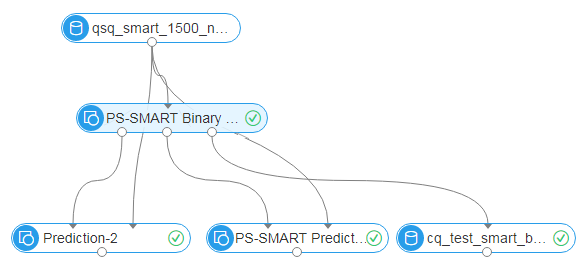As shown in the figure, a PS-SMART binary classification model is learned based on training data. The model has three output studs:

• Output model: offline model, which is connected to the unified prediction component. This model does not support output of leaf node numbers.

• Output model table: a binary table that is not readable. To ensure compatibility with the PS-SMART prediction component, the table provides outputs such as leaf node numbers and evaluation metrics. However, the output table has strict requirements on data formats, resulting in poor user experiences. It will be improved gradually or be replaced by another component.

• Output feature importance table: lists importance of each feature. Three importance types are supported (see parameter description).

### PAI command

Training

``PAI -name ps_smart``    -project algo_public``    -DinputTableName="smart_binary_input"``    -DmodelName="xlab_m_pai_ps_smart_bi_545859_v0"``    -DoutputTableName="pai_temp_24515_545859_2"``    -DoutputImportanceTableName="pai_temp_24515_545859_3"``    -DlabelColName="label"``    -DfeatureColNames="f0,f1,f2,f3,f4,f5"``    -DenableSparse="false"``    -Dobjective="binary:logistic"``    -Dmetric="error"``    -DfeatureImportanceType="gain"``    -DtreeCount="5";``    -DmaxDepth="5"``    -Dshrinkage="0.3"``    -Dl2="1.0"``    -Dl1="0"``    -Dlifecycle="3"``    -DsketchEps="0.03"``    -DsampleRatio="1.0"``    -DfeatureRatio="1.0"``    -DbaseScore="0.5"``    -DminSplitLoss="0"``

Prediction

``PAI -name prediction``    -project algo_public``    -DinputTableName="smart_binary_input";``    -DmodelName="xlab_m_pai_ps_smart_bi_545859_v0"``    -DoutputTableName="pai_temp_24515_545860_1"``    -DfeatureColNames="f0,f1,f2,f3,f4,f5"``    -DappendColNames="label,qid,f0,f1,f2,f3,f4,f5"``    -DenableSparse="false"``    -Dlifecycle="28"``

#### Parameter description

Data parameters

Command option Parameter Description Value range Required/Optional, default value
featureColNames Feature Column Name of the feature column selected from the input table for training The column name must be bigint or double type in dense format or string type in sparse KV format. If the sparse KV format is used, the keys and values must be numerical type data. Required
labelColName Label Column Name of the label column in the input table The column name can be either a string or a numerical value, but it can only be saved as a numerical value. For example, the value can be 0 or 1 for binary classification. Required
weightCol Weight Column You can specify a weight for each row of samples. Column name, numerical type (Optional) It is left blank by default.
enableSparse Sparse Format Whether data is in sparse format, in which key-value pairs are separated by spaces whereas keys and values are separated by colons, for example, `1:0.3 3:0.9` [true, false] (Optional) The default value is false.
inputTableName Name of the input table NA NA Required
modelName Name of the output model NA NA Required
outputImportanceTableName Output Feature Importance Table Name NA NA (Optional) It is left blank by default.
inputTablePartitions Input Table Partitions NA NA Optional, in the format of ds=1/pt=1
outputTableName Output Model Table Name The output table is an ODPS table that uses the binary format and is not readable. The self-contained prediction component of SMART can be used to provide output of leaf node numbers. true, false (Optional) The default value is false.
lifecycle Output Table Lifecycle NA Positive integer (Optional) The default value is 3.

Algorithm parameters

Command option Parameter Description Value range Required/Optional, default value
objective Objective Function Type The objective function type affects learning directly and must be selected correctly. Select “binary:logistic” for binary classification. Required
metric Evaluation Metric Type Evaluation metrics in the training set, which are output to stdout of the coordinator in a logview logloss, error, auc (Optional) It is left blank by default.
treeCount Number of Decision Trees Number of decision trees. The training time is in direct proportion to this number. Positive integer (Optional) The default value is 1.
maxDepth Maximum Decision Tree Depth Maximum depth of a decision tree, recommended value: 5 (a maximum of 32 leaf nodes) Positive integer, [1, 20] (Optional) The default value is 5.
sampleRatio Data Sampling Ratio Data sampling ratio for creating a weak learner to accelerate training when building each decision tree (0, 1] (Optional) The default value is 1.0, indicating that data sampling is disabled.
featureRatio Feature Sampling Ratio Feature sampling ratio for creating a weak learner to accelerate training when building each decision tree (0, 1] (Optional) The default value is 1.0, indicating that feature sampling is disabled.
l1 L1 Penalty Coefficient This parameter controls the number of leaf nodes. The larger the value is, the fewer the leaf nodes are. Set a larger value for overfitting. Non-negative real number (Optional) The default value is 0.
l2 L2 Penalty Coefficient This parameter controls distribution of leaf nodes. The larger the value is, the more evenly the leaf nodes are distributed.Set a larger value for overfitting. Non-negative real number (Optional) The default value is 1.0.
shrinkage Learning Rate (0, 1] (Optional) The default value is 0.3.
sketchEps Sketch Precision Threshold of the splitting point when creating a sketch. The number of bins is O (1.0/sketchEps). The smaller the value is, the more bins are divided. This value does not need to be adjusted under normal conditions. (0, 1) (Optional) The default value is 0.03.
minSplitLoss Minimum Split Loss Minimum split loss required for splitting a node. The larger the value is, the more conservatively the node splits. Non-negative real number (Optional) The default value is 0.
featureNum Feature Quantity Number of features or the largest feature ID. Specify this parameter for resource usage estimation. Positive integer Optional
baseScore Global Offset Original predicted values of all samples Real number (Optional) The default value is 0.5.
featureImportanceType Feature Importance Type Type of feature importance. “weight” indicates the number of times features are split. “gain” indicates information gain provided by the feature. “cover” indicates the number of samples that feature covers on the splitting node. “weight”, “gain”, “cover” (Optional) The default value is “gain”.

NOTE:

• Specify different values for the objective parameter in different learning models. On the binary classification web GUI, the objective function is automatically specified and hidden from users. On the command line interface, set the objective parameter to “binary:logistic”.
• Mappings between metrics and objective functions are: “logloss” for “negative loglikelihood for logistic regression”, “error” for “binary classification error”, and “auc” for “Area under curve for classification”.

#### Execution optimization

Command option Parameter Description Value range Required/Optional, default value
coreNum Number of Cores Number of cores used for computing. The larger the value is, the faster the computing algorithm runs. Positive integer (Optional) Cores are automatically assigned by default.
memSizePerCore Memory Size per Core (MB) Memory sized used by each core, where 1024 represents 1 GB memory Positive integer (Optional) Memory is automatically assigned by default.

#### Example

Prepare

Data generation

The following example generates data in dense format:

``drop table if exists smart_binary_input;``create table smart_binary_input lifecycle 3 as``select``*``from``(``select 0.72 as f0, 0.42 as f1, 0.55 as f2, -0.09 as f3, 1.79 as f4, -1.2 as f5, 0 as label from dual``union all``select 1.23 as f0, -0.33 as f1, -1.55 as f2, 0.92 as f3, -0.04 as f4, -0.1 as f5, 1 as label from dual``union all``select -0.2 as f0, -0.55 as f1, -1.28 as f2, 0.48 as f3, -1.7 as f4, 1.13 as f5, 1 as label from dual``union all``select 1.24 as f0, -0.68 as f1, 1.82 as f2, 1.57 as f3, 1.18 as f4, 0.2 as f5, 0 as label from dual``union all``select -0.85 as f0, 0.19 as f1, -0.06 as f2, -0.55 as f3, 0.31 as f4, 0.08 as f5, 1 as label from dual``union all``select 0.58 as f0, -1.39 as f1, 0.05 as f2, 2.18 as f3, -0.02 as f4, 1.71 as f5, 0 as label from dual``union all``select -0.48 as f0, 0.79 as f1, 2.52 as f2, -1.19 as f3, 0.9 as f4, -1.04 as f5, 1 as label from dual``union all``select 1.02 as f0, -0.88 as f1, 0.82 as f2, 1.82 as f3, 1.55 as f4, 0.53 as f5, 0 as label from dual``union all``select 1.19 as f0, -1.18 as f1, -1.1 as f2, 2.26 as f3, 1.22 as f4, 0.92 as f5, 0 as label from dual``union all``select -2.78 as f0, 2.33 as f1, 1.18 as f2, -4.5 as f3, -1.31 as f4, -1.8 as f5, 1 as label from dual``) tmp;``

The following figure shows the generated data.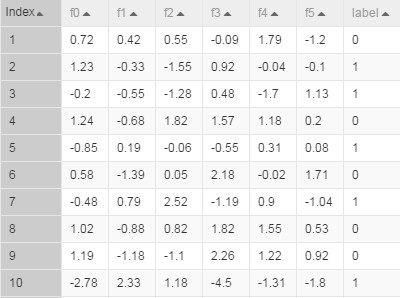The table contains six feature columns.

Training

Configure training data and the training component according to Prepare. Select the label column as the target column and columns f0, f1, f2, f3, f4, f5 as feature columns. The following figure shows the algorithm parameter settings page.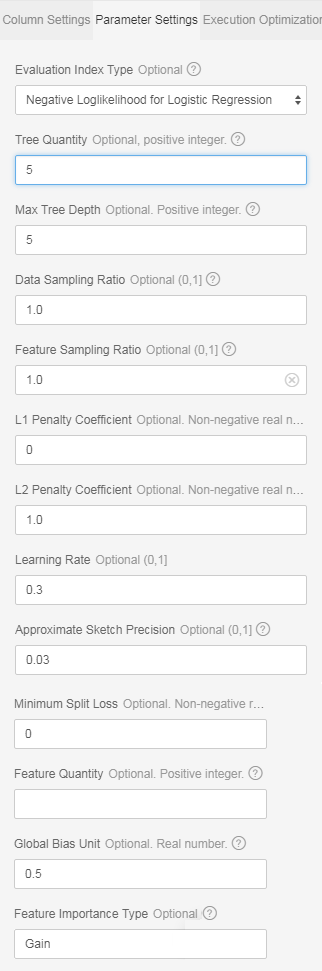You do not need to set the number of features because this number is calculated automatically by the algorithm. If you have a large number of features and want the algorithm to accurately estimate the amount of resources required, enter the actual number of features here.

To accelerate the training, you can set the number of cores on the execution optimization page. The larger the number is, the faster the algorithm runs. Generally, you do not need to enter the memory size per core because the algorithm can accurately estimate the memory size. In addition, the PS algorithm starts to run only when all hosts obtain resources. Therefore, you may need to wait for a longer time when the cluster is busy and requires many resources.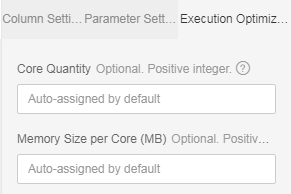You can view metrics output values in stdout of the coordinator in a logview (http link starting with “http://logview.odps.aliyun-inc.com:8080/logview“). One PS-SMART training job has multiple tasks; therefore, multiple logviews are available. Select the logview with logs starting with PS, as shown in the following figure.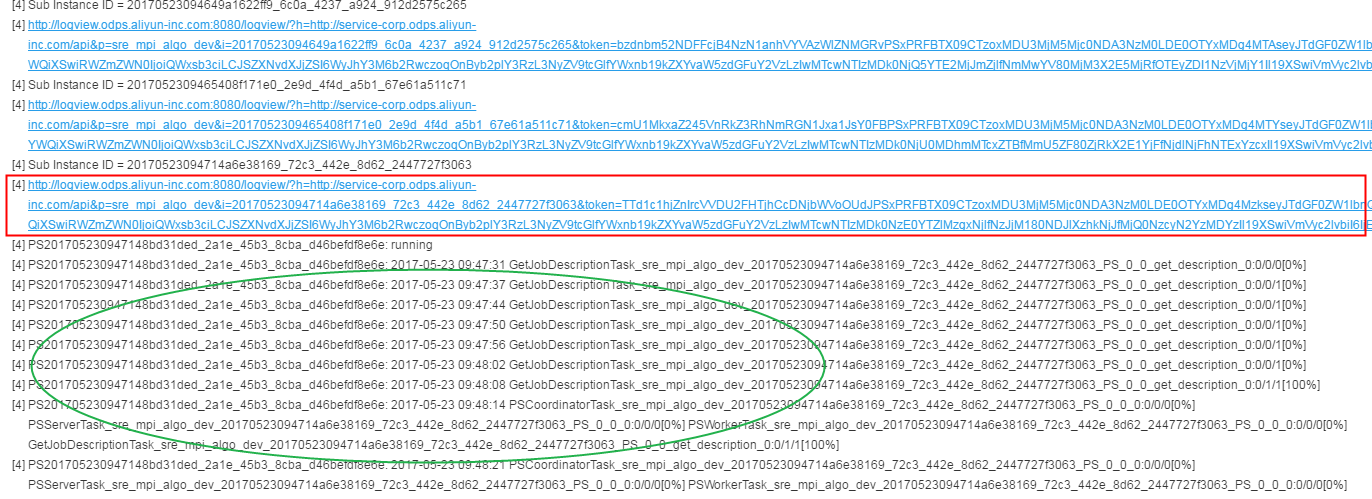The one in the red box is the logview of the PS job. You can identify different tasks by information in the green circle.

Then, perform operations in the logview according to the following figure.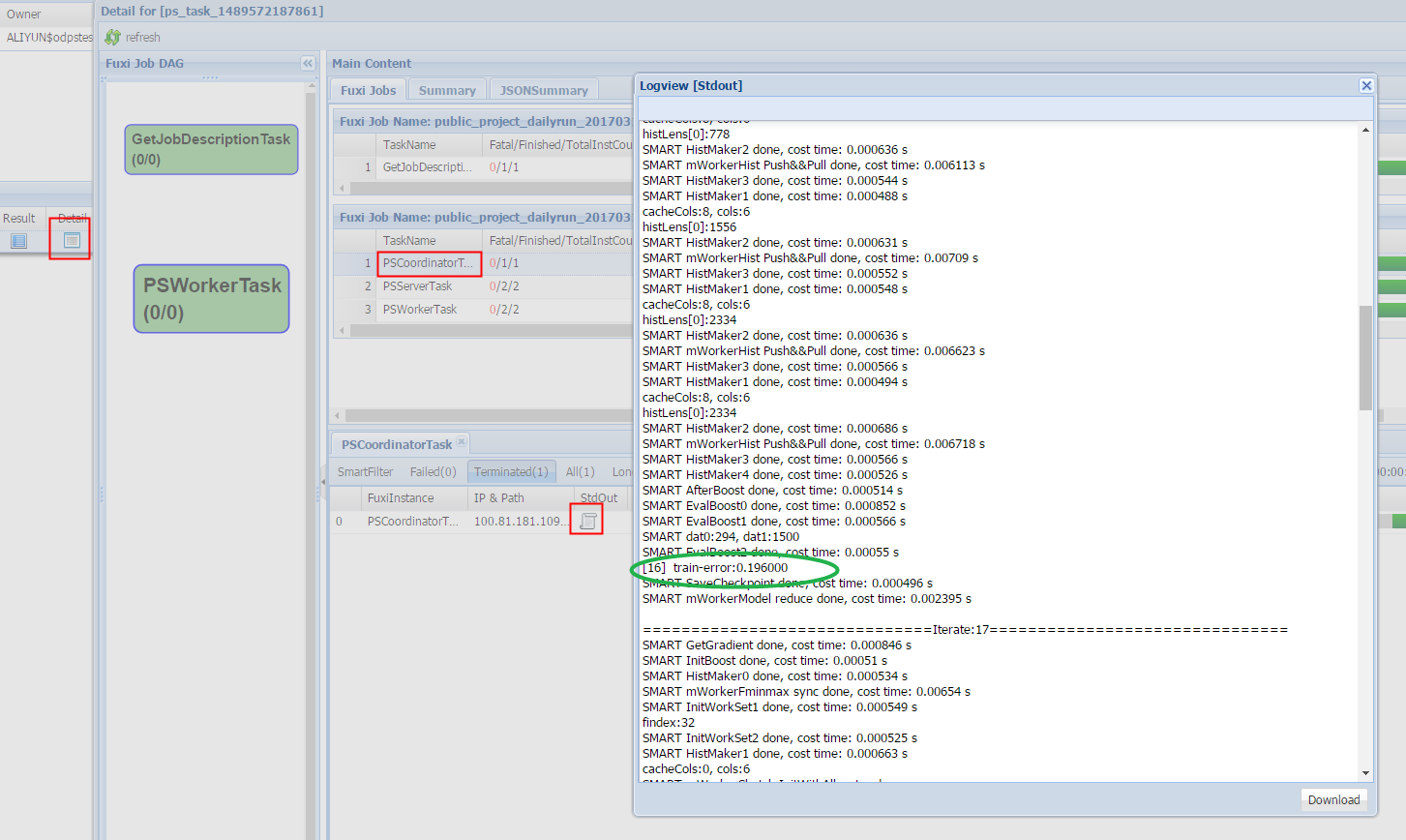Prediction

Use the unified prediction component

The output model obtained after training is saved in binary format and can be used for prediction. Configure the input model and test data for the prediction component according to Prepare and set parameters according to the following figure.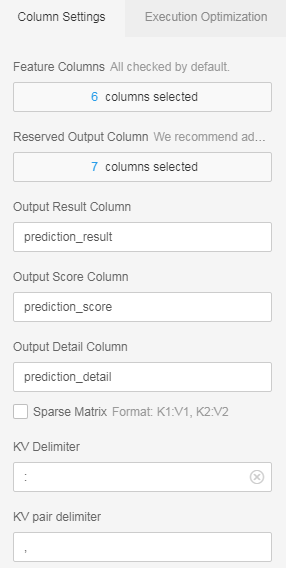If the dense format is used, you only need to select feature columns. (All columns are selected by default, and extra columns do not affect the prediction.) If the KV format is used, set the data format to sparse format and select a correct delimiter. In the SMART model, key-value pairs are separated by space characters. Therefore, the delimiter must be set to space or “\u0020” (escape expression of space).

The following figure shows the prediction result.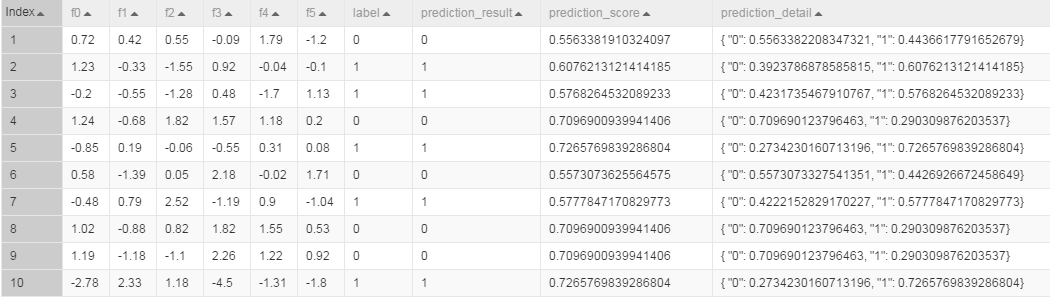In the “prediction_detail” column, the value 1 indicates a positive sample, and the value 0 indicates a negative sample. The values following 0 and 1 indicate probabilities of the corresponding classes.

Use the PS-SMART prediction component

The output model table obtained after training is saved in binary format and can be used by the PS-SMART prediction component for prediction. Configure the input model and test data for the prediction component according to Prepare and set parameters, including the data format, feature columns, target column, and number of classes. The ID column can only be a string type column other than feature columns and the target column. The loss function must be explicitly set to “binary:logistic”. The following figure shows the prediction result.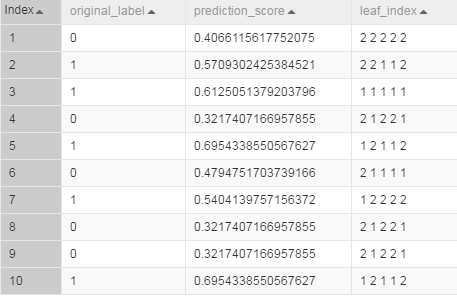The “prediction_score” column lists probabilities of predicted positive samples. A sample is predicted as a positive sample if its score is greater than 0.5. Otherwise, it is predicted as a negative sample. The “leaf_index” column lists the predicted leaf node numbers. Each sample has N numbers, where N is the number of decision trees. Each tree maps to a number, which indicates the number of the leaf node on this tree.

NOTE:

• The output model table is a binary table that is not readable. To ensure compatibility with the PS-SMART prediction component, the table provides outputs such as leaf node numbers and evaluation metrics.However, the output table has strict requirements on data formats, resulting in poor user experiences. It will be improved gradually or be replaced by another component.
• A string type column must be selected as the label column. You can enter character strings in the column but cannot leave it blank or enter Null in it. A feature column can be converted into the string type by using the data type conversion component and used as the label column.
• The loss function must be explicitly set to “binary:logistic”. By default, the loss function does not work.

View feature importance

To view feature importance, you can export the third output stud to an output table, or directly right-click in the PS-SMART component and choose View Data > Output Feature Importance Table. The following figure shows the output feature importance table.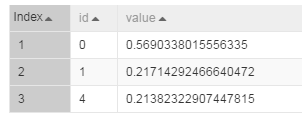In the table, the id column lists numbers of input features. In this example, the data is in dense format and input features are “f0, f1, f2, f3, f4, f5”. Therefore, ID 0 represents f0, and ID 4 represents f4. If the KV format is used, the IDs represent keys in key-value pairs. Each value indicates a feature importance type. The default value is “gain”, indicating the sum of information gains brought by a feature in the model. The preceding figure shows only three features because only these three features are used in split of trees. The importance of unused features is considered 0.

#### Important notes

• The target column in a PS-SMART binary classification model supports only numerical values (0 for negative samples and 1 for positive samples). Even if values in the ODPS table are strings, they are saved as numerical values. If the classification target is a type string similar to “Good” or “Bad”, convert it into 1 or 0.

• In the key-value format, feature IDs must be positive integers, and feature values must be real numbers. If feature IDs are character strings, use the serialization component to serialize them. If feature values are type character strings, perform engineering on the features, such as discretization.

• Although PS-SMART supports tasks with hundreds of thousands of features, such tasks consume many resources and run slowly. Therefore, we do not recommend such a large number of features. The GBDT algorithm is suitable for training with continuous features. Continuous type features require one-hot coding (to filter out infrequent features) before they can be used for training. Continuous numerical features can be used for training with the GBDT algorithm directly. Discretization is not recommended for numerical features.

• The PS-SMART algorithm applies randomness in many scenarios. For example, the data_sample_ratio and fea_sample_ratio items require data or feature sampling. In addition, the PS-SMART algorithm uses histograms to show similarity. When multiple workers run in a cluster in distributed mode, local sketches are merged to global sketches in a random order. Although different merging orders result in different tree structures, this does not bring great variation in the output model theoretically. Therefore, it is a normal situation if different results are obtained after the algorithm runs multiple times with the same data and same parameter settings.

## PS-SMART multiclass classification

PS stands for parameter server, which is used for online and offline training tasks of large-scale models. Scalable Multiple Additive Regression Tree (SMART) is an implementation of Gradient boosting decision tree (GBDT) on PS. PS-SMART can run training tasks containing up to tens of billions of samples and hundreds of thousands of features on thousands of nodes. It also supports failover to maintain a high stability. Additionally, PS-SMART supports various data formats, training targets, evaluation targets, output feature importance, and training acceleration (such as histogram similarity).

#### Quick StartAs shown in the figure, a PS-SMART multiclass classification model is learned based on training data.The model has three output studs:

• Output model: offline model, which is connected to the unified prediction component. This model does not support output of leaf node numbers.

• Output model table: a binary table that is not readable. To ensure compatibility with the PS-SMART prediction component, the table provides outputs such as leaf node numbers and evaluation metrics.However, the output table has strict requirements on data formats, resulting in poor user experiences. It will be improved gradually or be replaced by another component.

• Output feature importance table: lists importance of each feature. Three importance types are supported (see parameter description).

#### PAI command

Training

``PAI -name ps_smart``    -project algo_public``    -DinputTableName="smart_multiclass_input"``    -DmodelName="xlab_m_pai_ps_smart_bi_545859_v0"``    -DoutputTableName="pai_temp_24515_545859_2"``    -DoutputImportanceTableName="pai_temp_24515_545859_3"``    -DlabelColName="label"``    -DfeatureColNames="features"``    -DenableSparse="true"``    -Dobjective="multi:softprob"``    -Dmetric="mlogloss"``    -DfeatureImportanceType="gain"``    -DtreeCount="5";``    -DmaxDepth="5"``    -Dshrinkage="0.3"``    -Dl2="1.0"``    -Dl1="0"``    -Dlifecycle="3"``    -DsketchEps="0.03"``    -DsampleRatio="1.0"``    -DfeatureRatio="1.0"``    -DbaseScore="0.5"``    -DminSplitLoss="0"``

Prediction

``PAI -name prediction``    -project algo_public``    -DinputTableName="smart_multiclass_input";``    -DmodelName="xlab_m_pai_ps_smart_bi_545859_v0"``    -DoutputTableName="pai_temp_24515_545860_1"``    -DfeatureColNames="features"``    -DappendColNames="label,features"``    -DenableSparse="true"``    -DkvDelimiter=":"``    -Dlifecycle="28"``

#### Parameter description

Data parameters

Command option Parameter Description Value range Required/Optional, default value
featureColNames Feature Column Name of the feature column selected from the input table for training The column name must be bigint or double type in dense format or string type in sparse KV format. If the sparse KV format is used, the keys and values must be numerical type data. Required
labelColName Label Column Name of the label column in the input table The column name can be either a string or a numerical value, but it can only be saved as a numerical value. For multiclass classification, select the column name among 0, 1, 2, …, n-1, where n is the number of classes Required
weightCol Weight Column You can specify a weight for each row of samples. Column name, numerical type (Optional) It is left blank by default.
enableSparse Sparse Format Whether data is in sparse format, in which key-value pairs are separated by spaces whereas keys and values are separated by colons, for example, `1:0.3 3:0.9` [true, false] (Optional) The default value is false.
inputTableName Name of the input table NA NA Required
modelName Name of the output model NA NA Required
outputImportanceTableName Output Feature Importance Table Name NA NA (Optional) It is left blank by default.
inputTablePartitions Input Table Partitions NA NA Optional, in the format of ds=1/pt=1
outputTableName Output Model Table Name The output table is an ODPS table that uses the binary format and is not readable. The self-contained prediction component of SMART can be used to provide output of leaf node numbers. true, false (Optional) The default value is false.
lifecycle Output Table Lifecycle NA Positive integer (Optional) The default value is 3.

Algorithm parameters

Command option Parameter Description Value range Required/Optional, default value
classNum Number of Classes Number of classes in multiclass classification. If the number of classes is n, the label column name can be 0, 1, 2, …, or n-1 Integer greater than or equal to 3 Required
objective Objective Function Type The objective function type affects learning directly and must be selected correctly. Set it to “multi:softprob” for multiclass classification. Required
metric Evaluation Metric Type Evaluation metrics in the training set, which are output to stdout of the coordinator in a logview “mlogloss”,”merror” (Optional) It is left blank by default.
treeCount Number of Decision Trees Number of decision trees. The training time is in direct proportion to this number. Positive integer (Optional) The default value is 1.
maxDepth Maximum Decision Tree Depth Maximum depth of a decision tree, recommended value: 5 (a maximum of 32 leaf nodes) Positive integer, [1, 20] (Optional) The default value is 5.
sampleRatio Data Sampling Ratio Data sampling ratio for creating a weak learner to accelerate training when building each decision tree (0, 1] (Optional) The default value is 1.0, indicating that data sampling is disabled.
featureRatio Feature Sampling Ratio Feature sampling ratio for creating a weak learner to accelerate training when building each decision tree (0, 1] (Optional) The default value is 1.0, indicating that feature sampling is disabled.
l1 L1 Penalty Coefficient This parameter controls the number of leaf nodes. The larger the value is, the fewer the leaf nodes are.Set a larger value for overfitting. Non-negative real number (Optional) The default value is 0.
l2 L2 Penalty Coefficient This parameter controls distribution of leaf nodes. The larger the value is, the more evenly the leaf nodes are distributed.Set a larger value for overfitting. Non-negative real number (Optional) The default value is 1.0.
shrinkage Learning Rate (0, 1] (Optional) The default value is 0.3.
sketchEps Sketch Precision Threshold of the splitting point when creating a sketch. The number of bins is O (1.0/sketchEps).The smaller the value is, the more bins are divided. This value does not need to be adjusted under normal conditions. (0, 1) (Optional) The default value is 0.03.
minSplitLoss Minimum Split Loss Minimum split loss required for splitting a node. The larger the value is, the more conservatively the node splits. Non-negative real number (Optional) The default value is 0.
featureNum Feature Quantity Number of features or the largest feature ID.Specify this parameter for resource usage estimation. Positive integer Optional
baseScore Global Offset Original predicted values of all samples Real number (Optional) The default value is 0.5.
featureImportanceType Feature Importance Type Type of feature importance. “weight” indicates the number of times features are split. “gain” indicates information gain provided by the feature. “cover” indicates the number of samples that feature covers on the splitting node. “weight”, “gain”, “cover” (Optional) The default value is “gain”.

NOTE:

• Specify different values for the objective parameter in different learning models. On the multiclass classification web GUI, the objective function is automatically specified and hidden from users. On the command line interface, set the objective parameter to “multi:softprob”.

• Mappings between metrics and objective functions are: “mlogloss” for “multiclass negative log likelihood” and “merror” for “multiclass classification error”.

Execution optimization

Command option Parameter Description Value range Required/Optional, default value
coreNum Number of Cores Number of cores used for computing. The larger the value is, the faster the computing algorithm runs. Positive integer (Optional) Cores are automatically assigned by default.
memSizePerCore Memory Size per Core (MB) Memory sized used by each core, where 1024 represents 1 GB memory Positive integer (Optional) Memory is automatically assigned by default.

#### Example

Data generation

The following example uses the KV data format.

``drop table if exists smart_multiclass_input;``create table smart_multiclass_input lifecycle 3 as``select``*``from``(``select 2 as label, '1:0.55 2:-0.15 3:0.82 4:-0.99 5:0.17' as features from dual``    union all``select 1 as label, '1:-1.26 2:1.36 3:-0.13 4:-2.82 5:-0.41' as features from dual``    union all``select 1 as label, '1:-0.77 2:0.91 3:-0.23 4:-4.46 5:0.91' as features from dual``    union all``select 2 as label, '1:0.86 2:-0.22 3:-0.46 4:0.08 5:-0.60' as features from dual``    union all``select 1 as label, '1:-0.76 2:0.89 3:1.02 4:-0.78 5:-0.86' as features from dual``    union all``select 1 as label, '1:2.22 2:-0.46 3:0.49 4:0.31 5:-1.84' as features from dual``    union all``select 0 as label, '1:-1.21 2:0.09 3:0.23 4:2.04 5:0.30' as features from dual``    union all``select 1 as label, '1:2.17 2:-0.45 3:-1.22 4:-0.48 5:-1.41' as features from dual``    union all``select 0 as label, '1:-0.40 2:0.63 3:0.56 4:0.74 5:-1.44' as features from dual``    union all``select 1 as label, '1:0.17 2:0.49 3:-1.50 4:-2.20 5:-0.35' as features from dual``) tmp;``

The following figure shows the generated data.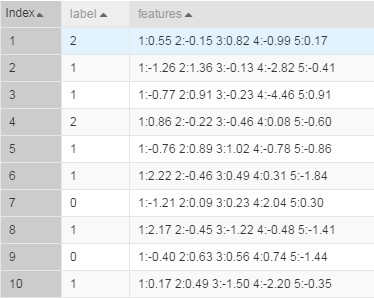The table contains five dimensions of features.

Training

Configure training data and the training component according to Prepare. Select the “label” column as the target column and “features” column as the feature column. The following figures show the data parameter settings page and algorithm parameter settings page.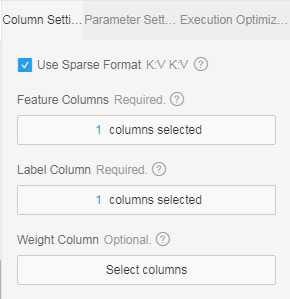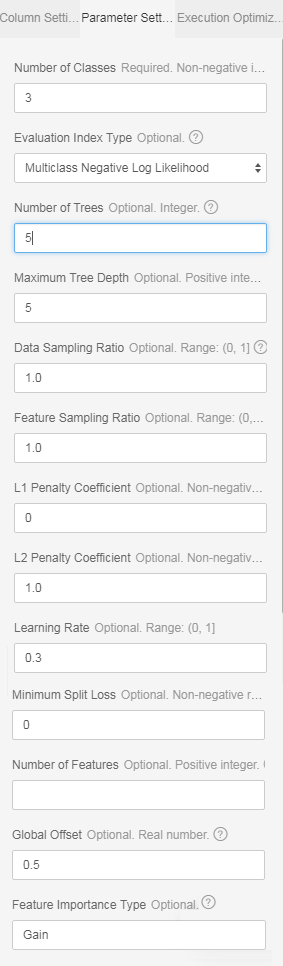You do not need to set the number of features because this number is calculated automatically by the algorithm.If you have a large number of features and want the algorithm to accurately estimate the amount of resources required, enter the actual number of features here.

To accelerate the training, you can set the number of cores on the execution optimization page. The larger the number is, the faster the algorithm runs.Generally, you do not need to enter the memory size per core because the algorithm can accurately estimate the memory size.In addition, the PS algorithm starts to run only when all hosts obtain resources. Therefore, you may need to wait for a longer time when the cluster is busy and requires many resources.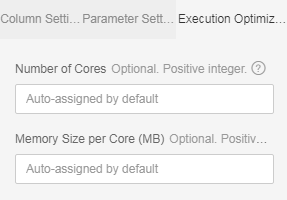You can view metrics output values in stdout of the coordinator in a logview (http link starting with “http://logview.odps.aliyun-inc.com:8080/logview“). One PS-SMART training job has multiple tasks; therefore, multiple logviews are available. Select the logview with logs starting with PS, as shown in the following figure.The one in the red box is the logview of the PS job. You can identify different tasks by information in the green circle.

Then, perform operations in the logview according to the following figure.Prediction

Use the unified prediction component

The output model obtained after training is saved in binary format and can be used for prediction.Configure the input model and test data for the prediction component according to Prepare and set parameters according to the following figure.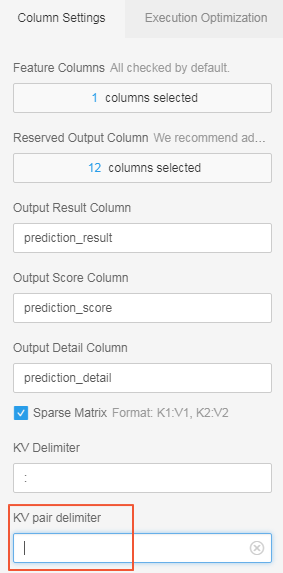If the dense format is used, you only need to select feature columns. (All columns are selected by default, and extra columns do not affect the prediction.)If the KV format is used, set the data format to sparse format and select a correct delimiter.In the SMART model, key-value pairs are separated by space characters. Therefore, the delimiter must be set to space or “\u0020” (escape expression of space).

The following figure shows the prediction result.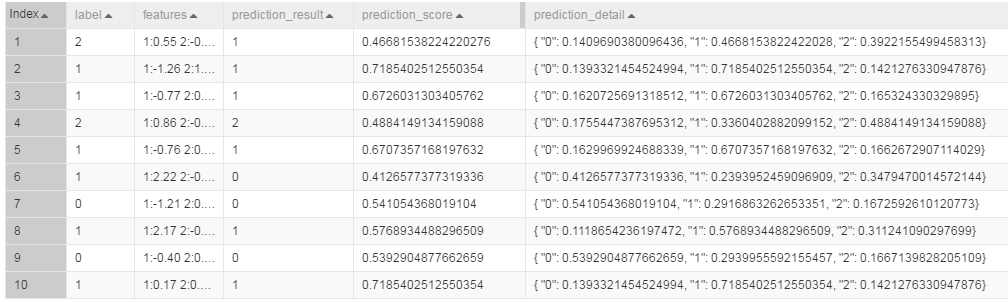In the “prediction_detail” column, values 0, 1, and 2 indicate classes, and the values following them indicate probabilities of the corresponding classes. The “predict_result” column lists the selected classes with the highest probability, and the “predict_score” column lists the probability of each selected class.

Use the PS-SMART prediction component

The output model table obtained after training is saved in binary format and can be used by the PS-SMART prediction component for prediction.Configure the input model and test data for the prediction component according to Prepare and set parameters, including the data format, feature columns, target column, and number of classes. The ID column can only be a string type column other than feature columns and the target column. The loss function must be explicitly set to “multi:softprob”. The following figure shows the prediction result.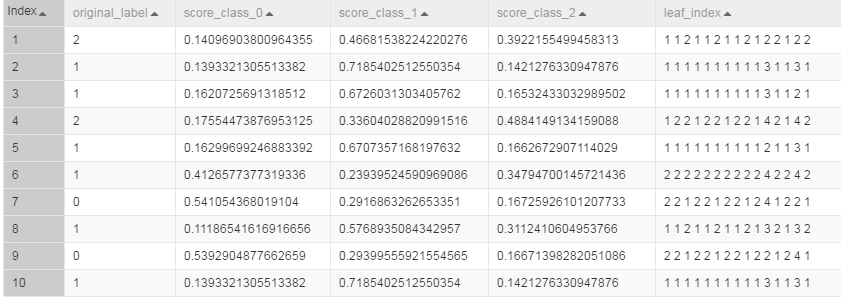The “score_class_k” columns list probabilities of class k. The class with the highest probability is the predicted class. The “leaf_index” column lists the predicted leaf node numbers. Each sample has N*M numbers, where N is the number of decision trees, and M is the number of classes. In this example, each sample has 15 numbers (5*3 = 15).Each tree maps to a number, which indicates the number of the leaf node on this tree.

NOTE:

• The output model table is a binary table that is not readable. To ensure compatibility with the PS-SMART prediction component, the table provides outputs such as leaf node numbers and evaluation metrics.However, the output table has strict requirements on data formats, resulting in poor user experiences. It will be improved gradually or be replaced by another component.

• A string type column must be selected as the label column. You can enter character strings in the column but cannot leave it blank or enter Null in it. A feature column can be converted into the string type by using the data type conversion component and used as the label column.

• The loss function must be explicitly set to “multi:softprob”. By default, the loss function does not work.

View feature importance

To view feature importance, you can export the third output stud to an output table, or directly right-click in the PS-SMART component and choose View Data > Output Feature Importance Table. The following figure shows the output feature importance table.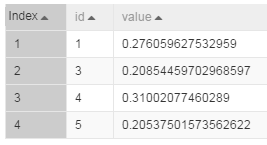If the values in the id column are input feature numbers and the KV format is used, the IDs represent keys in key-value pairs. If the dense format is used and input features are “f0, f1, f2, f3, f4, f5”, ID 0 represents f0, and ID 4 represents f4.Each value indicates a feature importance type. The default value is “gain”, indicating the sum of information gains brought by a feature in the model. The preceding figure shows only four features because only these four features are used in the split of trees. The importance of unused features is considered 0.

#### Important notes

• The target column in a PS-SMART multiclass classification model supports only positive integer IDs (class numbers are 0, 1, 2, …, n-1, where n is the number of classes). Even if the values in the ODPS table are strings, they are saved as numerical values. If the classification target is a type string similar to “Good”, “Medium”, or “Bad”, convert it into a numerical value (0, 1, 2, …, n-1).

• In the key-value format, feature IDs must be positive integers, and feature values must be real numbers.If feature IDs are character strings, use the serialization component to serialize them.If feature values are type character strings, perform engineering on the features, such as discretization.

• Although PS-SMART supports tasks with hundreds of thousands of features, such tasks consume many resources and run slowly. Therefore, we do not recommend such a large number of features.The GBDT algorithm is suitable for training with continuous features. Continuous type features require one-hot coding (to filter out infrequent features) before they can be used for training. Continuous numerical features can be used for training with the GBDT algorithm directly. Discretization is not recommended for numerical features.

• The PS-SMART algorithm applies randomness in many scenarios. For example, the data_sample_ratio and fea_sample_ratio items require data or feature sampling.In addition, the PS-SMART algorithm uses histograms to show similarity. When multiple workers run in a cluster in distributed mode, local sketches are merged to global sketches in a random order. Although different merging orders result in different tree structures, this does not bring great variation in the output model theoretically.Therefore, it is a normal situation if different results are obtained after the algorithm runs multiple times with the same data and same parameter settings.

## PS-SMART regression

PS stands for parameter server, which is used for online and offline training tasks of large-scale models. Scalable Multiple Additive Regression Tree (SMART) is an implementation of Gradient boosting decision tree (GBDT) on PS. PS-SMART can run training tasks containing up to tens of billions of samples and hundreds of thousands of features on thousands of nodes. It also supports failover to maintain a high stability. Additionally, PS-SMART supports various data formats, training targets, evaluation targets, output feature importance, and training acceleration (such as histogram similarity).

#### Quick StartAs shown in the figure, a PS-SMART regression model is learned based on training data.The model has three output studs:

• Output model: offline model, which is connected to the unified prediction component. This model does not support output of leaf node numbers.

• Output model table: a binary table that is not readable. To ensure compatibility with the PS-SMART prediction component, the table provides outputs such as leaf node numbers and evaluation metrics.However, the output table has strict requirements on data formats, resulting in poor user experiences. It will be improved gradually or be replaced by another component.

• Output feature importance table: lists importance of each feature. Three importance types are supported (see parameter description).

#### PAI command

Training

``PAI -name ps_smart``    -project algo_public``    -DinputTableName="smart_regression_input"``    -DmodelName="xlab_m_pai_ps_smart_bi_545859_v0"``    -DoutputTableName="pai_temp_24515_545859_2"``    -DoutputImportanceTableName="pai_temp_24515_545859_3"``    -DlabelColName="label"``    -DfeatureColNames="features"``    -DenableSparse="true"``    -Dobjective="reg:linear"``    -Dmetric="rmse"``    -DfeatureImportanceType="gain"``    -DtreeCount="5";``    -DmaxDepth="5"``    -Dshrinkage="0.3"``    -Dl2="1.0"``    -Dl1="0"``    -Dlifecycle="3"``    -DsketchEps="0.03"``    -DsampleRatio="1.0"``    -DfeatureRatio="1.0"``    -DbaseScore="0.5"``    -DminSplitLoss="0"``

Prediction

``PAI -name prediction``    -project algo_public``    -DinputTableName="smart_regression_input";``    -DmodelName="xlab_m_pai_ps_smart_bi_545859_v0"``    -DoutputTableName="pai_temp_24515_545860_1"``    -DfeatureColNames="features"``    -DappendColNames="label,features"``    -DenableSparse="true"``    -Dlifecycle="28"``

#### Parameter description

Data parameters

Command option Parameter Description Value range Required/Optional, default value
featureColNames Feature Column Name of the feature column selected from the input table for training The column name must be bigint or double type in dense format or string type in sparse KV format. If the sparse KV format is used, the keys and values must be numerical type data. Required
labelColName Label Column Name of the label column in the input table The column name can be either a string or a numeric value, but it can only be a numerical value when saved in internal storage. For example, the value can be 0 or 1 for regression. Required
weightCol Weight Column You can specify a weight for each row of samples. Column name, numerical type (Optional) It is left blank by default.
enableSparse Sparse Format Whether data is in sparse format, in which key-value pairs are separated by spaces whereas keys and values are separated by colons, for example, “1:0.3 3:0.9” true, false (Optional) The default value is false.
inputTableName Name of the input table NA NA Required
modelName Name of the output model NA NA Required
outputImportanceTableName Output Feature Importance Table Name NA NA (Optional) It is left blank by default.
inputTablePartitions Input Table Partitions NA NA Optional, in the format of ds=1/pt=1
outputTableName Output Model Table Name The output table is an ODPS table that uses the binary format and is not readable. The self-contained prediction component of SMART can be used to provide output of leaf node numbers. true, false (Optional) The default value is false.
lifecycle Output Table Lifecycle NA Positive integer (Optional) The default value is 3.

Algorithm parameters

Command option Parameter Description Value range Required/Optional, default value
objective Objective Function Type The objective function type affects learning directly and must be selected correctly. Multiple loss functions can be used for regression. See the notes below. NA (Required) The default type is linear regression.
metric Evaluation Metric Type Evaluation metrics in the training set, which must be corresponding to the objective function type and are output to stdout of the coordinator in a logview. See the notes and samples below. NA (Optional) It is left blank by default.
treeCount Number of Decision Trees Number of decision trees. The training time is in direct proportion to this number. Positive integer (Optional) The default value is 1.
maxDepth Maximum Decision Tree Depth Maximum depth of a decision tree, recommended value: 5 (a maximum of 32 leaf nodes) Positive integer, [1, 20] (Optional) The default value is 5.
sampleRatio Data Sampling Ratio Data sampling ratio for creating a weak learner to accelerate training when building each decision tree (0, 1] (Optional) The default value is 1.0, indicating that data sampling is disabled.
featureRatio Feature Sampling Ratio Feature sampling ratio for creating a weak learner to accelerate training when building each decision tree (0, 1] (Optional) The default value is 1.0, indicating that feature sampling is disabled.
l1 L1 Penalty Coefficient This parameter controls the number of leaf nodes. The larger the value is, the fewer the leaf nodes are.Set a larger value for overfitting. Non-negative real number (Optional) The default value is 0.
l2 L2 Penalty Coefficient This parameter controls distribution of leaf nodes. The larger the value is, the more evenly the leaf nodes are distributed.Set a larger value for overfitting. Non-negative real number (Optional) The default value is 1.0.
shrinkage Learning Rate (0, 1] (Optional) The default value is 0.3.
sketchEps Sketch Precision Threshold of the splitting point when creating a sketch. The number of bins is O (1.0/sketchEps).The smaller the value is, the more bins are divided. This value does not need to be adjusted under normal conditions. (0, 1) (Optional) The default value is 0.03.
minSplitLoss Minimum Split Loss Minimum split loss required for splitting a node. The larger the value is, the more conservatively the node splits. Non-negative real number (Optional) The default value is 0.
featureNum Feature Quantity Number of features or the largest feature ID.Specify this parameter for resource usage estimation. Positive integer Optional
baseScore Global Offset Original predicted values of all samples Real number (Optional) The default value is 0.5.
featureImportanceType Feature Importance Type Type of feature importance. “weight” indicates the number of times features are split. “gain” indicates information gain provided by the feature. “cover” indicates the number of samples that feature covers on the splitting node. “weight”, “gain”, “cover” (Optional) The default value is “gain”.
tweedieVarPower Tweedie Distribution Index Tweedie distribution index indicating the relationship between the variance and mean. \$Var(y) ~ E(y)^tweedie_variance_power\$ (1, 2) (Optional) The default value is 1.5.

NOTE:

• Specify different values for the objective parameter in different learning models. The regression web GUI provides multiple objective functions, which are described as follows:
``reg:linear (Linear regression) Note: The range of label numbers is (-∞, +∞).``reg:logistic (Logistic regression) Note: The range of label numbers is [0, 1].``count:poisson (Poisson regression for count data, output mean of poisson distribution) Note: Label numbers must be greater than 0.``reg:gamma (Gamma regression for modeling insurance claims severity, or for any outcome that might be [gamma-distributed](https://en.wikipedia.org/wiki/Gamma_distribution#Applications)) Note: Label numbers must be greater than 0.``reg:tweedie (Tweedie regression for modeling total loss in insurance, or for any outcome that might be [Tweedie-distributed](https://en.wikipedia.org/wiki/Tweedie_distribution#Applications).) Note: Label numbers must be greater than 0.``
• Metrics for these objective functions are:
``rmse (rooted mean square error, corresponding to objective reg:linear)``mae (mean absolute error, corresponding to objective reg:linear)``poisson-nloglik (negative loglikelihood for poisson regression, corresponding to objective count:poisson)``gamma-deviance (Residual deviance for gamma regression, corresponding to objective reg:gamma)``gamma-nloglik (Negative log-likelihood for gamma regression, corresponding to objective reg:gamma)``tweedie-nloglik (tweedie-nloglik@1.5, negative log-likelihood for Tweedie regression, at a specified value of the tweedie_variance_power parameter)``

Execution optimization

Command option Parameter Description Value range Required/Optional, default value
coreNum Number of Cores Number of cores used for computing. The larger the value is, the faster the computing algorithm runs. Positive integer (Optional) Cores are automatically assigned by default.
memSizePerCore Memory Size per Core (MB) Memory sized used by each core, where 1024 represents 1 GB memory Positive integer (Optional) Memory is automatically assigned by default.

#### Example

Data generation

The following example uses the sparse KV data format.

``drop table if exists smart_regression_input;``create table smart_regression_input as``select``*``from``(``select 2.0 as label, '1:0.55 2:-0.15 3:0.82 4:-0.99 5:0.17' as features from dual``    union all``select 1.0 as label, '1:-1.26 2:1.36 3:-0.13 4:-2.82 5:-0.41' as features from dual``    union all``select 1.0 as label, '1:-0.77 2:0.91 3:-0.23 4:-4.46 5:0.91' as features from dual``    union all``select 2.0 as label, '1:0.86 2:-0.22 3:-0.46 4:0.08 5:-0.60' as features from dual``    union all``select 1.0 as label, '1:-0.76 2:0.89 3:1.02 4:-0.78 5:-0.86' as features from dual``    union all``select 1.0 as label, '1:2.22 2:-0.46 3:0.49 4:0.31 5:-1.84' as features from dual``    union all``select 0.0 as label, '1:-1.21 2:0.09 3:0.23 4:2.04 5:0.30' as features from dual``    union all``select 1.0 as label, '1:2.17 2:-0.45 3:-1.22 4:-0.48 5:-1.41' as features from dual``    union all``select 0.0 as label, '1:-0.40 2:0.63 3:0.56 4:0.74 5:-1.44' as features from dual``    union all``select 1.0 as label, '1:0.17 2:0.49 3:-1.50 4:-2.20 5:-0.35' as features from dual``) tmp;``

The following figure shows the generated data.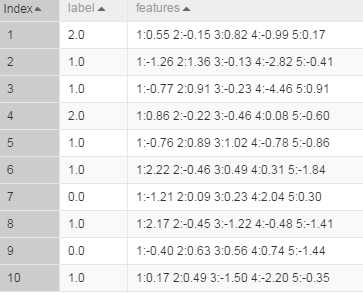In the table, feature IDs are numbered starting from 1, and the maximum feature ID is 5.

Training

Select the “label” column as the target column and “features” column as the feature column. The following figure shows the algorithm parameter settings page for linear regression.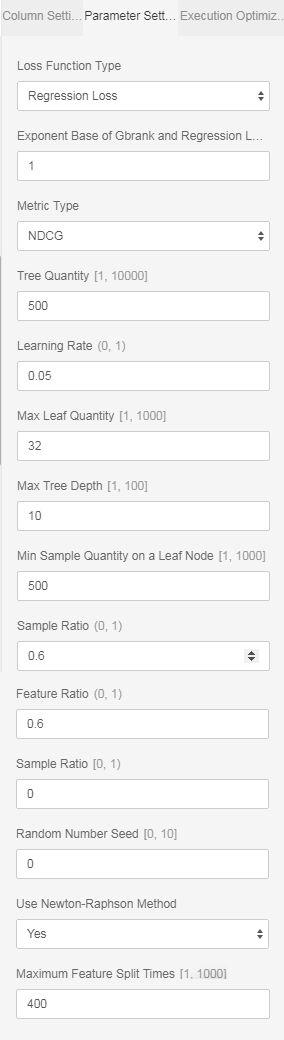You do not need to set the number of features because this number is calculated automatically by the algorithm.If you have a large number of features and want the algorithm to accurately estimate the amount of resources required, enter the actual number of features here.

To accelerate the training, you can set the number of cores on the execution optimization page. The larger the number is, the faster the algorithm runs.Generally, you do not need to enter the memory size per core because the algorithm can accurately estimate the memory size.In addition, the PS algorithm starts to run only when all hosts obtain resources. Therefore, you may need to wait for a longer time when the cluster is busy and requires many resources.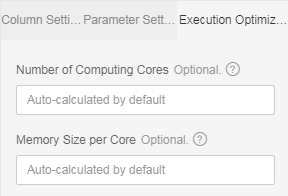You can view metrics output values in stdout of the coordinator in a logview (http link starting with “http://logview.odps.aliyun-inc.com:8080/logview“). One PS-SMART training job has multiple tasks; therefore, multiple logviews are available. Select the logview with logs starting with PS, as shown in the following figure.The one in the red box is the logview of the PS job. You can identify different tasks by information in the green circle.

Then, perform operations in the logview according to the following figure.Prediction

Use the unified prediction component

The output model obtained after training is saved in binary format and can be used for prediction.Configure the input model and test data for the prediction component according to Prepare and set parameters according to the following figure.If the dense format is used, you only need to select feature columns. (All columns are selected by default, and extra columns do not affect the prediction.)If the KV format is used, set the data format to sparse format and select a correct delimiter.In the SMART model, key-value pairs are separated by space characters. Therefore, the delimiter must be set to space or “\u0020” (escape expression of space).

The following figure shows the prediction result.The “predict_result” column lists the predicted values.

Use the PS-SMART prediction component

The output model table obtained after training is saved in binary format and can be used by the PS-SMART prediction component for prediction.Configure the input model and test data for the prediction component according to Prepare and set parameters, including the data format, feature columns, target column, and number of classes. The ID column can only be a string type column other than feature columns and the target column. The loss function must be explicitly set to the objective function used for training.The following figure shows the prediction result.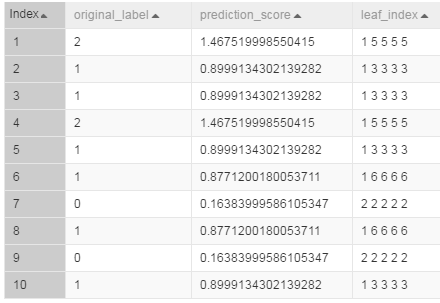The “prediction_score” column lists the predicted values. The “leaf_index” column lists the predicted leaf node numbers. Each sample has N numbers, where N is the number of decision trees.Each tree maps to a number, which indicates the number of the leaf node on this tree.

NOTE:

• The output model table is a binary table that is not readable. To ensure compatibility with the PS-SMART prediction component, the table provides outputs such as leaf node numbers and evaluation metrics.However, the output table has strict requirements on data formats, resulting in poor user experiences. It will be improved gradually or be replaced by another component.

• A string type column must be selected as the label column. You can enter character strings in the column but cannot leave it blank or enter Null in it. A feature column can be converted into the string type by using the data type conversion component and used as the label column.

• The loss function must be explicitly set to the objective function used for training. By default, the loss function does not work.

View feature importance

To view feature importance, you can export the third output stud to an output table, or directly right-click in the PS-SMART component and choose View Data > Output Feature Importance Table. The following figure shows the output feature importance table.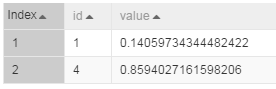If the values in the id column are input feature numbers and the KV format is used, the IDs represent keys in key-value pairs.If the dense format is used and input features are “f0, f1, f2, f3, f4, f5”, ID 0 represents f0, and ID 4 represents f4.Each value indicates a feature importance type. The default value is “gain”, indicating the sum of information gains brought by a feature in the model. The preceding figure shows only two features because only these two features are used in split of trees. The importance of unused features is considered 0.

#### Important notes

• The target column in a PS-SMART regression model supports only numerical values. Even if values in the ODPS table are strings, they are saved as numerical values.

• In the key-value format, feature IDs must be positive integers, and feature values must be real numbers.If feature IDs are character strings, use the serialization component to serialize them.If feature values are type character strings, perform engineering on the features, such as discretization.

• Although PS-SMART supports tasks with hundreds of thousands of features, such tasks consume many resources and run slowly. Therefore, we do not recommend such a large number of features.The GBDT algorithm is suitable for training with continuous features. Continuous type features require one-hot coding (to filter out infrequent features) before they can be used for training. Continuous numerical features can be used for training with the GBDT algorithm directly. Discretization is not recommended for numerical features.

• The PS-SMART algorithm applies randomness in many scenarios. For example, the data_sample_ratio and fea_sample_ratio items require data or feature sampling.In addition, the PS-SMART algorithm uses histograms to show similarity. When multiple workers run in a cluster in distributed mode, local sketches are merged to global sketches in a random order. Although different merging orders result in different tree structures, this does not bring great variation in the output model theoretically.Therefore, it is a normal situation if different results are obtained after the algorithm runs multiple times with the same data and same parameter settings.

## PS linear regression

Linear regression is a classic regression algorithm used to analyze the linear relationship between a dependent variable and multiple independent variables. A parameter server (PS) is used to run online and offline training tasks of large-scale models. Parameter servers can use over a hundred billion rows of samples to train tens of billions of feature models at high efficiency. The PS linear regression model can run training tasks with hundreds of billions of samples and billions of features, and supports L1 and L2 regular expressions. To run tasks of larger scales, contact the author of the algorithm.

#### Quick Start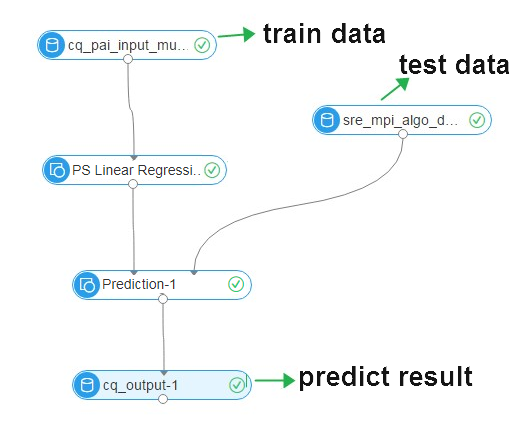### PAI command

Training

``PAI -name ps_linearregression``    -project algo_public``    -DinputTableName="lm_test_input"``    -DmodelName="linear_regression_model"``    -DlabelColName="label"``    -DfeatureColNames="features"``    -Dl1Weight=1.0``    -Dl2Weight=0.0``    -DmaxIter=100``    -Depsilon=1e-6``    -DenableSparse=true``

Prediction

``drop table if exists logistic_regression_predict;``PAI -name prediction``    -DmodelName="linear_regression_model"``    -DoutputTableName="linear_regression_predict"``    -DinputTableName="lm_test_input"``    -DappendColNames="label,features"``    -DfeatureColNames="features"``    -DenableSparse=true``

#### Parameter description

Data parameters

Command option Parameter Description Value range Required/Optional, default value
featureColNames Feature Columns Feature columns selected from the input table for training The column names must be bigint or double type in dense format or string type in sparse KV format. Required
labelColName Label Column Name of the label column in the input table The column name must be a numerical value (bigint or double type). Required
enableSparse Sparse Format If the sparse KV format is used, do not use feature ID 0. We recommend that you number feature IDs starting from 1. true, false (Optional) The default value is false.
itemDelimiter KV Pair Delimiter Delimiter used between key-value pairs when data in the input table is in sparse format NA (Optional) The default delimiter is space.
kvDelimiter Key and Value Delimiter Delimiter used between keys and values when data in the input table is in sparse format NA (Optional) The default delimiter is colon.
inputTableName Name of the input table NA NA Required
modelName Name of the output model NA NA Required
inputTablePartitions Input Table Partitions NA NA Optional, in the format of ds=1/pt=1
enableModelIo Output to Offline Model When this parameter is set to false, output data is exported to the ODPS table, where you can view weights of models true, false (Optional) The default value is true.

Algorithm parameters

Command option Parameter Description Value range Required/Optional, default value
l1Weight L1 weight L1 regularization coefficient. The larger the value is, the less zeros a model has.Set a larger value for overfitting. Non-negative real number (Optional) The default value is 1.0.
l2Weight L2 weight L2 regularization coefficient. The larger the value is, the smaller the model parameter absolute values are.Set a larger value for overfitting. Non-negative real number (Optional) The default value is 0.
maxIter Maximum Iterations Maximum number of L-BFGS/OWL-QN iterations. The value 0 indicates that the number of iterations is unlimited. Non-negative integer (Optional) The default value is 100.
epsilon Minimum Convergence Deviation Mean value of the relative loss change rate in ten iterations, which is used as the condition for determining whether to terminate the optimization algorithm. The smaller the value is, the more strict the condition is and the longer the algorithm runs. Real number between 0 and 1 (Optional) The default value is 1.0e-06.
modelSize Largest Feature ID Largest feature ID among all features (feature dimension). It can be larger than the actual largest feature ID. The larger the value is, the higher the memory usage is. If you leave this parameter blank, the system starts an SQL task to calculate the largest feature ID automatically. Non-negative integer (Optional) The default value is 0.

Both the maximum iterations and minimum convergence deviation determine when the algorithm stops. If both the parameters are set, the algorithm stops when either condition is triggered.

Execution optimization

Command option Parameter Description Value range Required/Optional, default value
coreNum Number of Cores Number of cores used for computing. The larger the value is, the faster the computing algorithm runs. Positive integer (Optional) Cores are automatically assigned by default.
memSizePerCore Memory Size per Core (MB) Memory sized used by each core, where 1024 represents 1 GB memory Positive integer (Optional) Memory is automatically assigned by default. Generally, you do not need to set this parameter because the algorithm can accurately estimate the memory size required.

#### Example

Data generation

The following example uses the sparse KV data format.

``drop table if exists lm_test_input;``create table lm_test_input as``select``*``from``(``select 2 as label, '1:0.55 2:-0.15 3:0.82 4:-0.99 5:0.17' as features from dual``    union all``select 1 as label, '1:-1.26 2:1.36 3:-0.13 4:-2.82 5:-0.41' as features from dual``    union all``select 1 as label, '1:-0.77 2:0.91 3:-0.23 4:-4.46 5:0.91' as features from dual``    union all``select 2 as label, '1:0.86 2:-0.22 3:-0.46 4:0.08 5:-0.60' as features from dual``    union all``select 1 as label, '1:-0.76 2:0.89 3:1.02 4:-0.78 5:-0.86' as features from dual``    union all``select 1 as label, '1:2.22 2:-0.46 3:0.49 4:0.31 5:-1.84' as features from dual``    union all``select 0 as label, '1:-1.21 2:0.09 3:0.23 4:2.04 5:0.30' as features from dual``    union all``select 1 as label, '1:2.17 2:-0.45 3:-1.22 4:-0.48 5:-1.41' as features from dual``    union all``select 0 as label, '1:-0.40 2:0.63 3:0.56 4:0.74 5:-1.44' as features from dual``    union all``select 1 as label, '1:0.17 2:0.49 3:-1.50 4:-2.20 5:-0.35' as features from dual``) tmp;``

The following figure shows the generated data.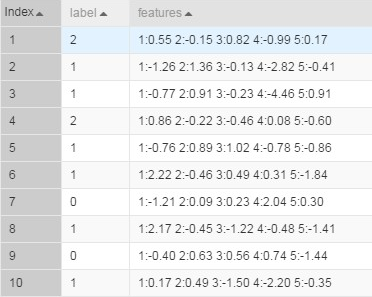In the table, feature IDs are numbered starting from 1, and the maximum feature ID is 5.

Training

Configure training data and the training component according to Prepare. Select the “label” column as the target column and “features” column as the feature column. Select the sparse data format.The following figure shows the algorithm parameter settings page.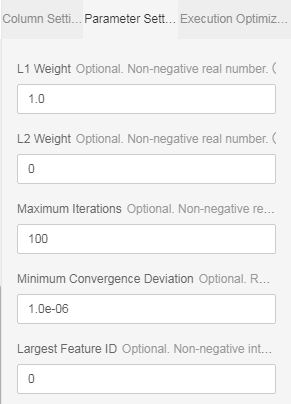You can retain the default value 0 of the largest feature ID. The algorithm can start an SQL task to calculate the largest feature ID automatically. If you do not want to start the SQL task, enter a value greater than 5. This value indicates the number of feature columns in dense format and indicates the largest feature ID in KV format.

To accelerate the training, you can set the number of cores on the execution optimization page. The larger the number is, the faster the algorithm runs.Generally, you do not need to enter the memory size per core because the algorithm can accurately estimate the memory size.In addition, the PS algorithm starts to run only when all hosts obtain resources. Therefore, you may need to wait for a longer time when the cluster is busy and requires many resources.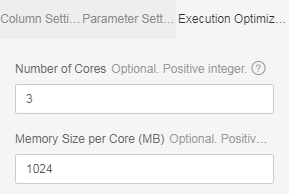Prediction

The model obtained after training is saved in binary format and can be used for prediction.Configure the input model and test data for the prediction component according to Prepare and set parameters according to the following figure.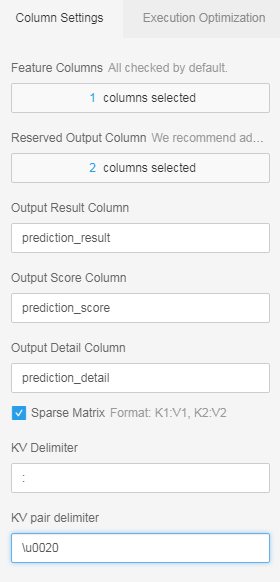Select the KV format used for training and set a correct delimiter. When the KV format is used, key-value pairs are separated by space characters. Therefore, the delimiter must be set to space or “\u0020” (escape expression of space).

The following figure shows the prediction result.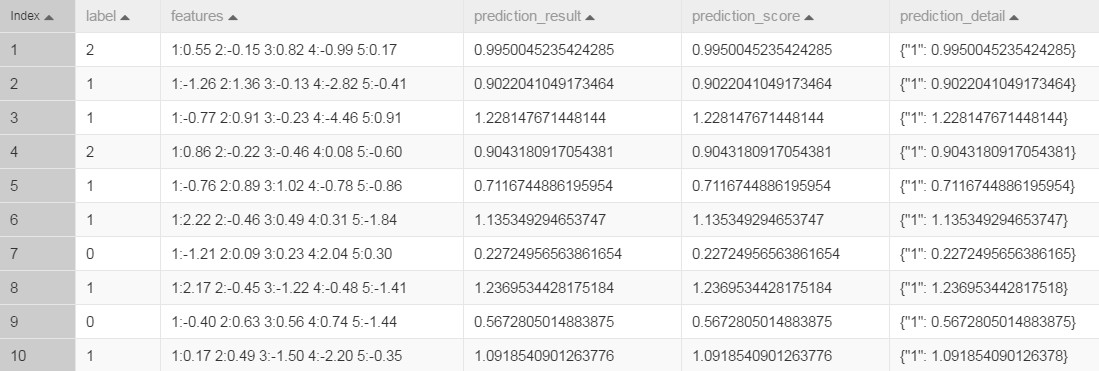You only need to view the predict_result column.

#### Important notes

In the key-value format, feature IDs must be positive integers, and feature values must be real numbers.If feature IDs are character strings, use the serialization component to serialize them.If feature values are type character strings, perform engineering on the features, such as discretization.

## Clustering model evaluation

This component evaluates clustering models based on metrics and icons.

#### PAI command

``PAI -name cluster_evaluation``    -project algo_public``    -DinputTableName=pai_cluster_evaluation_test_input``    -DselectedColNames=f0,f3``    -DmodelName=pai_kmeans_test_model``    -DoutputTableName=pai_ft_cluster_evaluation_out;``

#### Parameter description

Parameter Description Value range Required/Optional, default value/act
inputTableName Input table Table name Required
selectedColNames Names of the columns used for evaluation in the input table, which are separated by commas. The column names must be the same as feature names saved in the model. Column names (Optional) All columns in the input table are selected by default.
inputTablePartitions Partitions used for evaluation in the input table, in the format of name1=value1/name2=value2. Multiple partition names are separated by commas. NA (Optional) All partitions are selected by default.
enableSparse Whether data in the input table is in sparse format true, false Optional, default: false
itemDelimiter Delimiter used between key-value pairs when data in the input table is in sparse format NA Optional, default: space
kvDelimiter Delimiter used between keys and values when data in the input table is in sparse format NA Optional, default: colon
modelName Name of the input clustering model Model name Required
outputTableName Name of the output table Table name Required
lifecycle (Optional) Lifecycle of the output table Positive integer No lifecycle

#### Evaluation formula

The Calinski-Harabasz metric is also known as the variance ratio criterion (VRC). It is defined as follows: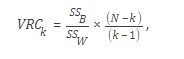•is the inter-cluster variance.

•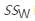is the intra-cluster variance.

• N is the total number of records, and k is the number of cluster centers.

•is defined as follows: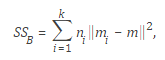• k is the number of the cluster centers.
•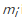is the center of cluster i, and m is the mean of input data.
•is defined as follows:• k is the number of cluster centers, and x is a data point.
•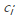indicates cluster i.
•indicates the center of cluster i.

#### Example

Test data

``create table if not exists pai_cluster_evaluation_test_input  as``select * from``(``  select 1 as id, 1 as f0,2 as f3 from dual``  union all``  select 2 as id, 1 as f0,3 as f3 from dual``  union all``  select 3 as id, 1 as f0,4 as f3 from dual``  union all``  select 4 as id, 0 as f0,3 as f3 from dual``  union all``  select 5 as id, 0 as f0,4 as f3 from dual``)tmp;``

Build a clustering model

``PAI -name kmeans``    -project algo_public``    -DinputTableName=pai_cluster_evaluation_test_input``    -DselectedColNames=f0,f3``    -DcenterCount=3``    -Dloop=10``    -Daccuracy=0.00001``    -DdistanceType=euclidean``    -DinitCenterMethod=random``    -Dseed=1``    -DmodelName=pai_kmeans_test_model``    -DidxTableName=pai_kmeans_test_idx``

PAI command

``PAI -name cluster_evaluation``    -project algo_public``    -DinputTableName=pai_cluster_evaluation_test_input``    -DselectedColNames=f0,f3``    -DmodelName=pai_kmeans_test_model``    -DoutputTableName=pai_ft_cluster_evaluation_out;``

Output description

Output table outputTableName, with the following fields:

column name comment
count Total number of records
centerCount Number of cluster centers
calinhara Calinski Harabasz metric Evaluation formula
clusterCounts Total number of points in each cluster

Display on the Machine Learning Platform For AI web GUI: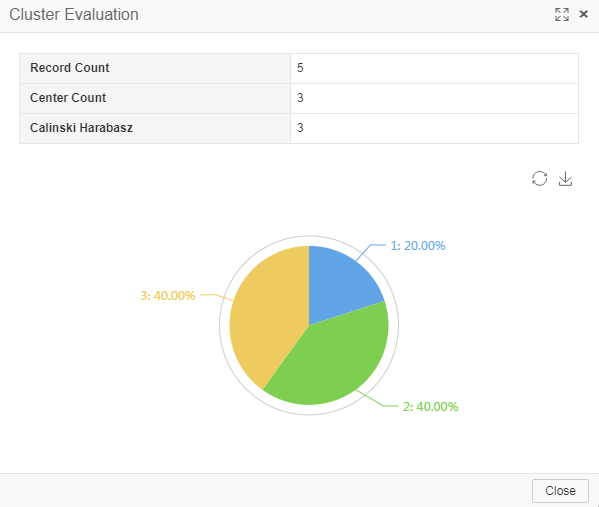PaiWeb-Pipeline: